# Filters and Ringing: Part 3

Now we’ve seen that if we have a filter that results in either a peak or a dip in the magnitude response, we’ll also result in the signal ringing in time. We’ve also seen that the frequency of the ringing is the centre frequency of the filter. Now let’s dig a little deeper into the behaviour of that ringing; or, more specifically its decay characteristics.

We’ll repeat the process from Part 2: measure the impulse response of a peaking filter where Fc = 1 kHz, gain = +12 dB, and Q = 2. However, this time I’ll look at the time response with a different scaling. Instead of plotting the linear value over time, I’ll convert each instantaneous value to dB and plot that. This looks like Figure 1.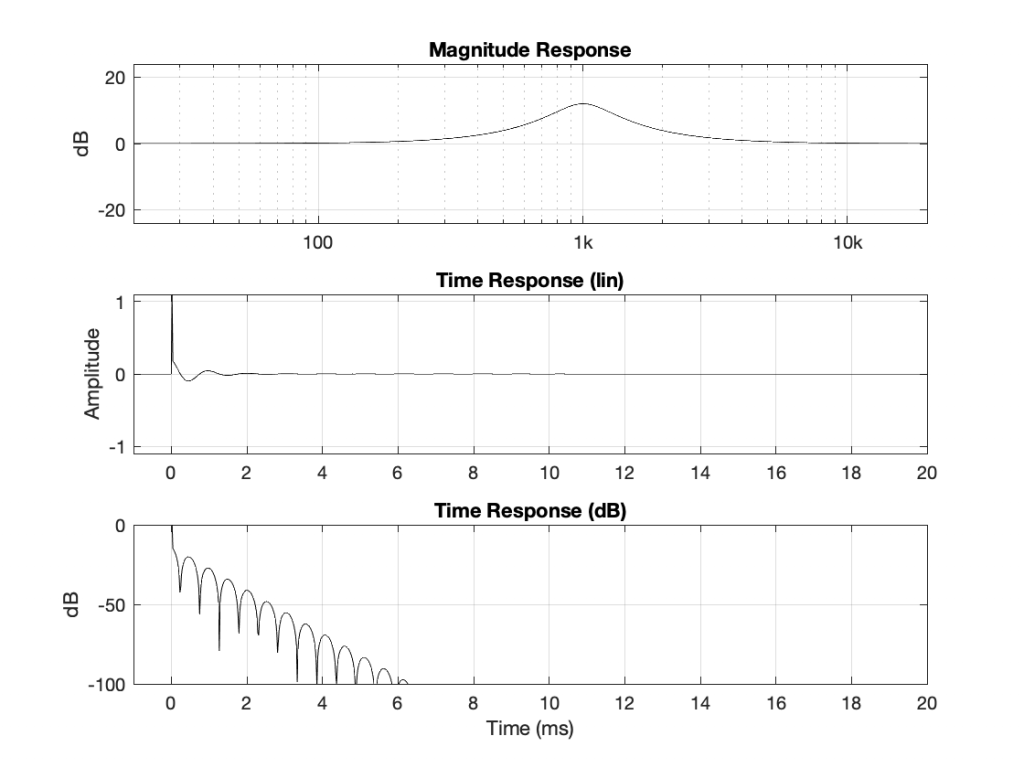Fig 1. The same filter from Part 1, but now I’m plotting the impulse response on an instantaneous decibel scale.

The important thing to notice here is that, when I plot the instantaneous amplitude in decibels (in other words, on a logarithmic scale), the decay is a straight line with a slope.

Let’s get two things out of the way here. This isn’t really decibels, because decibels requires some time averaging. Also, I’m actually plotting the absolute value of the impulse response in a decibel scale, because if I try to calculate the log of a negative number, things get ugly. This means that the math I’m actually using to create the bottom plot is

20 * log10(abs(signal))

If I draw a line across the tops of the bumps in that plot, I can look at the decay of the filter’s ringing as in Figure 2.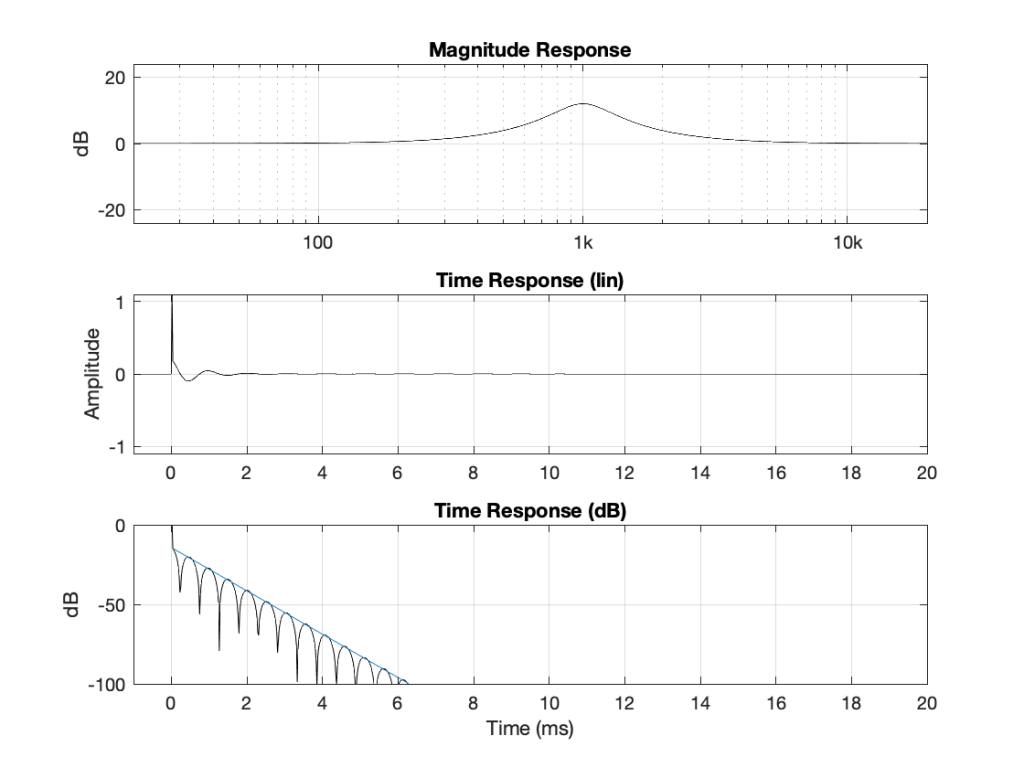Fig 2. The blue line shows the decay rate of the filter’s ringing. In this particular case, the decay is about -1360 dB per second.

For this filter, the decay rate of the ringing is -1360 dB per second (which is very fast). Let’s change some parameters and see what happens.

If I increase the gain of the filter without changing the Fc or the Q, I get the following:

I could plot lots more of these so that you start to see a pattern, but I’ll jump to the punch lines and you can use the plots above to check that things make sense.

If I have a filter that is using a definition of Q = Fc / BW (where BW is the distance between the -3 dB points down from the maximum), then:

• Changing the gain does not change the rate of the decay (all least, as long as it’s a boost, according to what we’ve seen so far…)
• Changing the Q will change the slope of the decay inversely proportionally if we’re measuring the slope in dB/sec. For example, if I multiply the Q by 2, the ringing decays twice as slowly. If I multiply the Q by 10, the ringing will take 10 times longer to decay to the same level.
• Changing the frequency will change the slope of the decay proportionally if we’re measuring the slope in dB/sec. For example, if I multiply the frequency by 2, the ringing will decay twice as fast.

Let’s talk about the last of these first, since it’s the easiest to understand conceptually. In the plots above, I’m showing the time in seconds. So, the higher the frequency, the more cycles I’m showing in the same plot. However, if I were plotting time in cycles of the cosine wave instead, the slope would be the same regardless of frequency.

In other words, the level of the ringing decays by the same amount per number of cycles of the cosine wave.

This is why, if you count the number of “bumps” in the dB plots in Figure 2 and 5, you’ll see that they are the same number. It takes about 12 cycles to get down to -100 dB, but the shorter the cycles (because the frequency is higher) the faster you get there when measuring in seconds. If the X-axis were not “Time in milliseconds”, but “Time in periods of the centre frequency” instead, then the slopes would be identical in Figures 2 and 5.

# Filters and Ringing: Part 2

## Rocks, Guitars, and Children

If you throw a rock into a pond on a windless day, you’ll see the ripples moving away in an expanding circle from the place where the rock hit. The ripples are places on the water where the water is either higher or lower than where it was before you hit the rock. The water itself only moves up and down, but the waves expand sideways. (You can see this if there is something floating on the water, for example – it bobs up and down as the waves go by.)

A similar thing happens when you pluck a guitar string. The point where your finger plucked is the same as the point where the rock landed in the water, and waves radiate away from that place on the string in two directions (because there are only two directions to travel in on a string: this way and that way). However, when those waves reach the end of the string, they reflect and come back in the opposite direction.

In both cases, the water and the guitar string, the wave has some speed at which it travels. It’s slow enough on the water for you to watch it, but it’s much too fast on a guitar string. In fact, it’s so fast that, when you pluck it, the wave travels to the end of the string, reflects in the opposite direction, hits the other end of the string, reflects again, and gets back to where you plucked it in about 1/82nd of a second if it’s the low E string. Since the wave doesn’t stop there – it keeps going, repeating the back-and-forth journey along the length of the string every 1/82nd of a second, then we hear a note with a fundamental frequency of 82 Hz (82 cycles per second): a low E.

That ringing that happens on the guitar string will happen no matter how you start the movement on it. You could hit the string with a chopstick, you could just thump the side of the guitar with your fist, you could even stand next to the guitar and cough loudly. All of these things will “inject” energy into the string, causing it to move, and the wave starts banging back and forth.

The rate of repetition is dependent on two things: the length of the string and the speed of the wave. The speed of the wave is dependent on two things: the mass of the string (e.g. how heavy is 1 m of it?) and the tension (how tightly is it stretched?) Increase the tension, and you increase the speed of the wave. Decrease the mass and you increase the speed of the wave. Increase the speed of the wave, and the repetition takes less time, so you hear a higher note.

That frequency at which the string will naturally ring is called a resonance. A child on a swing will go back and forth at the same rate (number of times per second) no matter how gently or forcefully you push them – apply energy, and the system will resonate.

Now, let’s think about that push of the child, the rock hitting the water, or the pluck of the guitar string. All of those things are a short injection of energy: a kind of impulse, and the way the child, the water, or the string behaves afterwards is its impulse response – how it responds to that impulse.

But here’s a strange thing to consider. This means that the note (the frequency) that you hear from the guitar string was one of the many frequencies in the initial pluck itself.

So, another way to think of this is that, by plucking the string, you inject a signal with all frequencies in it, and all of those frequencies decay (“die away”) very quickly except for one.

Okay, okay, if we’re going to be pedantic, I should be including not only the fundamental frequency but all of the additional harmonics; typically multiples of that frequency. But we don’t need to complicate things with the truth at the moment…

## What does this have to do with filters?

From a “big picture” point of view, a guitar string is a filter. I feed in some signal (the pluck) and I get out a modified version of that signal (the note ringing). From the same perspective, a filter in an equaliser is the same: I feed in a signal (music) and I get out a modified version of it (the same music, but slightly louder at 1 kHz, for example). What’s interesting is that the two things basically work the same way.

Let’s take the example of the filter at the end of Part 1: a peaking filter with a boost of 12 dB at 1 kHz, with a Q of 2. If I feed in a sine wave (which only contains energy at 1 frequency) at a very low frequency (say, 100 Hz or lower) then the level of the output will equal that of the input. If I do the same with a very high frequency (say, 10 kHz) then the level of the output will also equal that of the input. However, if I feed in a sine wave at 1 kHz, the output will be 4 times louder than the input (+12 dB = 4 time the amplitude because 20*log10(4) = 12-ish).

At some other frequency around 1 kHz, I’ll get a different answer. However, this is a VERY long and tedious way to measure the magnitude response of the filter. Another option is to measure its impulse response.

If I feed the input of the filter with an impulse (which is a sound that contains all frequencies at the same level, as we saw in Part 1), and look at the filters output in time, it might look like this:

Notice that the impulse looks like an impulse at Time = 0, but then something extra happens afterwards – like a guitar string ringing in time. If I zoom in vertically and look at the same plot, it will look like Figure 3.

And if we zoom in horizontally as well, it will look like this.

So, as you can see there, it’s almost as if we kept the impulse, and then just added a cosine wave with a period (a repetition time) of 1 ms, starting at Time = 0 and decaying over time. In fact, that’s exactly what the filter does.

## Time response to Frequency response

The excuse I gave above for sending an impulse through the filter (instead of sine waves) was that this will be a faster way to measure its response. The time response of the filter is already done. We can see that in the figures above. But how do we see the filter’s frequency response? This is done using a clever bit of math called a Fourier Transform, which lets you take a signal in time, and analyse its content by frequency. I won’t explain that here, but if you’re interested in how it works, you can start by reading this.

If I take the total impulse response (also known as a time response measurement) of the filter: in other words, I send in an impulse, I record the output and don’t stop recording until the ringing has decayed to a level low enough that I no longer care (for the purposes of this discussion, at least). Then, I do a Fourier Transform of the recording, I get something like Figure 5.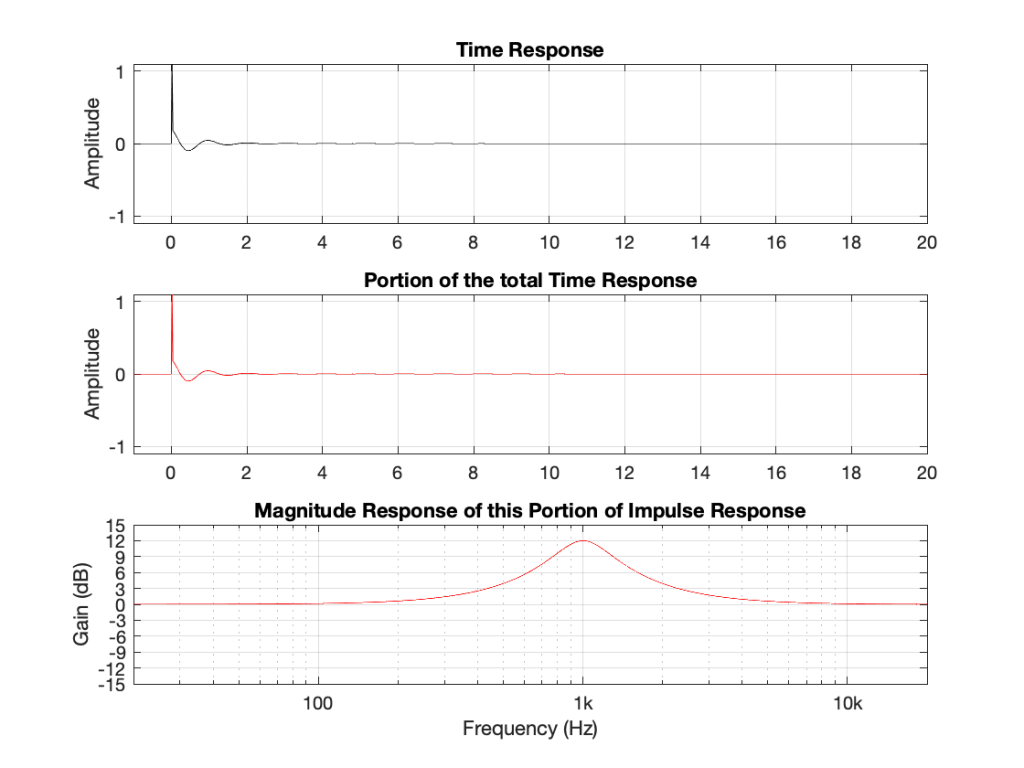Fig 5. In this example, the “portion” of the time response that I’ve used is the entire time response. Bear with me.

There is no new information in Figure 5. It’s just a setup for Figures 6 and 7.

Let’s now start slicing up the time response selectively to see what frequencies are contained in the output of the filter at what time. We’ll start by just taking the first and second samples of the impulse at the output, shown in Figure 6.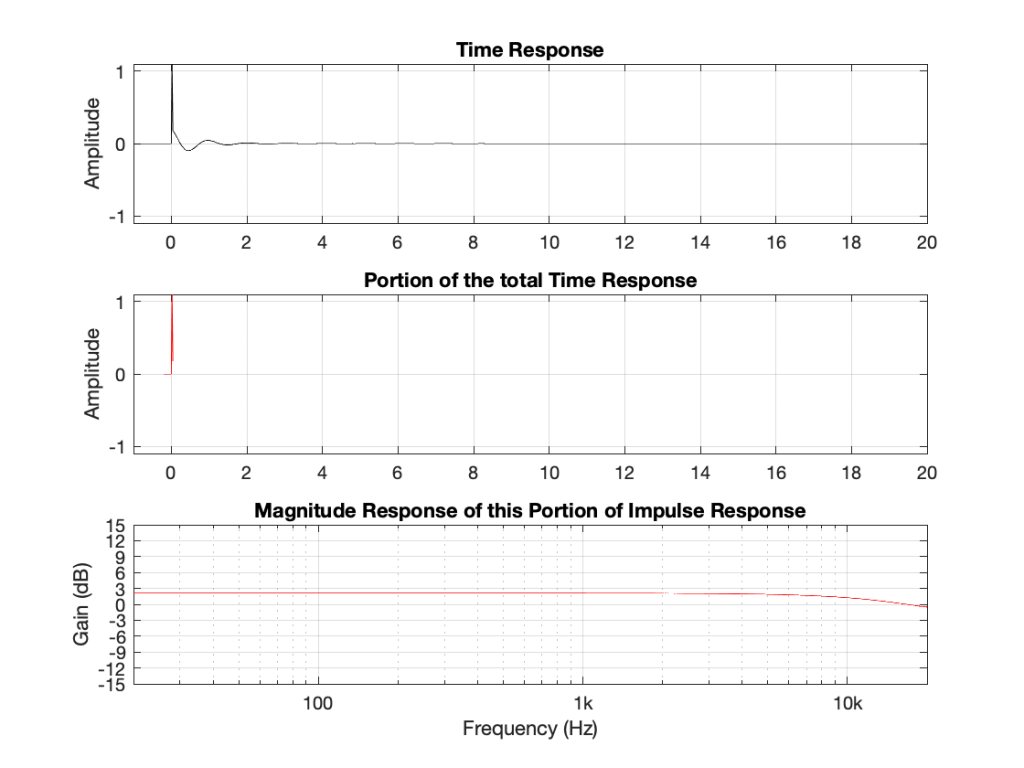Fig 6. The magnitude response is a measurement of ONLY the first 2 samples of the impulse, which are shown in the middle plot.

As you can see in Figure 6, if I remove the ringing that comes after the impulse, then the response of the signal has an almost-flat magnitude response and a gain of about 2 dB or so. This should not come as a surprise, since it’s almost an impulse. The only real difference between the portion that I’ve used and a real impulse is that the second value is not 0. So far so good…

Let’s look at the remainder of the time response. This is shown in Figure 7.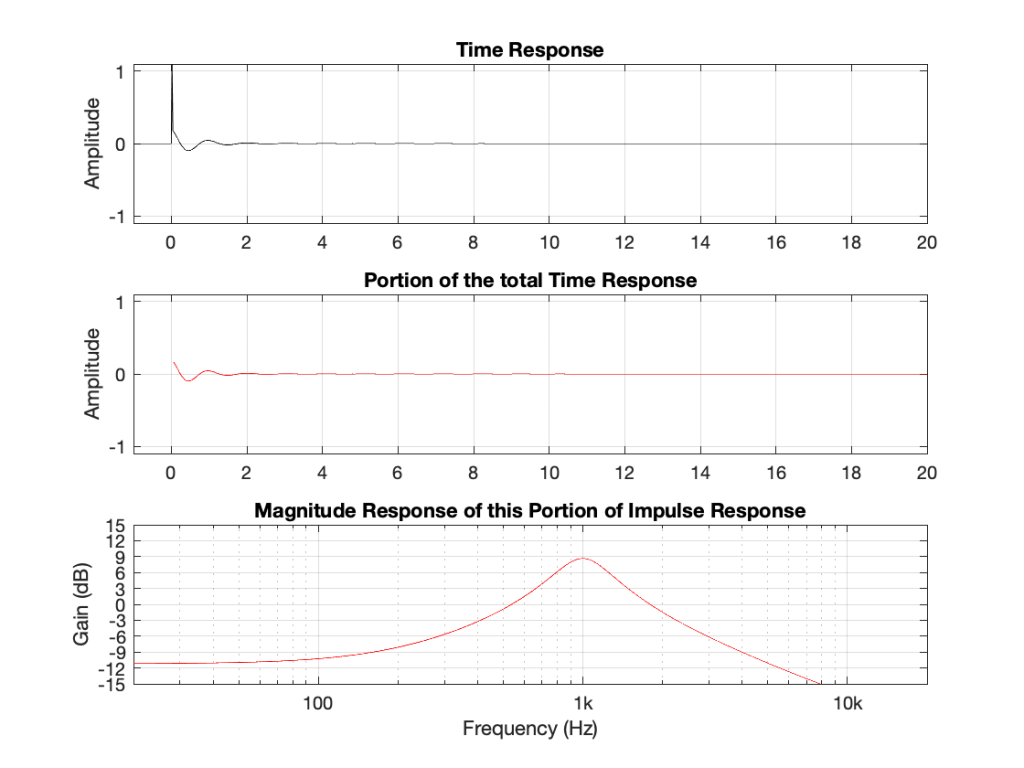Fig 7. The magnitude response of the remaining portion of the time response, omitting the initial onset of the impulse.

Figure 7 shows something interesting. We see the response of a band-pass filter with a centre frequency of 1 kHz, and a gain of 9 dB, which is the response of the filter after the initial impulse has passed.

## What does this all mean!?

If we leave out one important thing for now, this means that a peaking filter that has a boost of 12 dB, an Fc of 1 kHz and a Q of 2 is actually the sum of two things:

• a through-put with a little gain (about 1 dB)
• a bandpass filter with a gain of about 9 dB

This is, in essence, true. You can create a peaking filter by summing a bandpass filter to a through-put. However, an important point to realise here is that the band pass signal essentially comes after the onset of the signal. In Part 3, we’ll talk about whether this is a problem – or, more accurately, when this might be a problem. For now, however, I’ll throw one more example at you.

Up to now, we’ve only looked at the example of a peaking filter with a boost. What happens when the filter has a cut instead?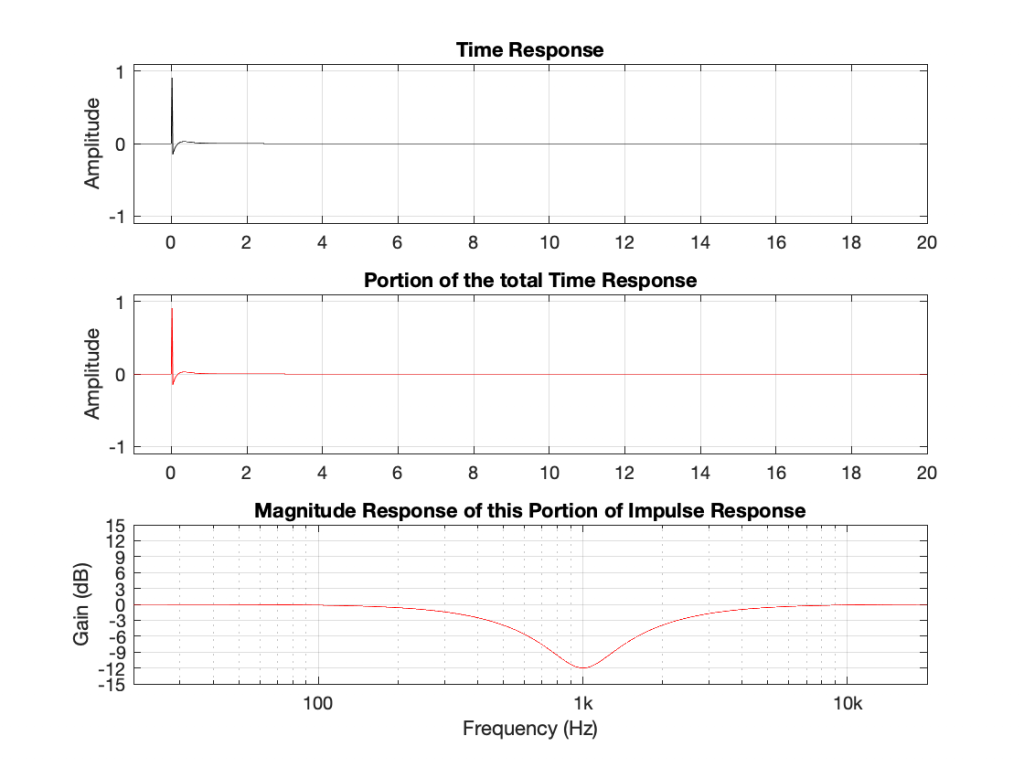Fig 8. The time and magnitude responses of a dip filter where Fc = 1 kHz, Gain = -12 dB and Q = 2.

Notice that a dip filter also rings in time after the initial impulse, but decays much faster than the equivalent boost. (I’ll have to be a bit more careful about my use of the word “equivalent”, actually – but I’ll straighten that out at the end of the series. To be continued…)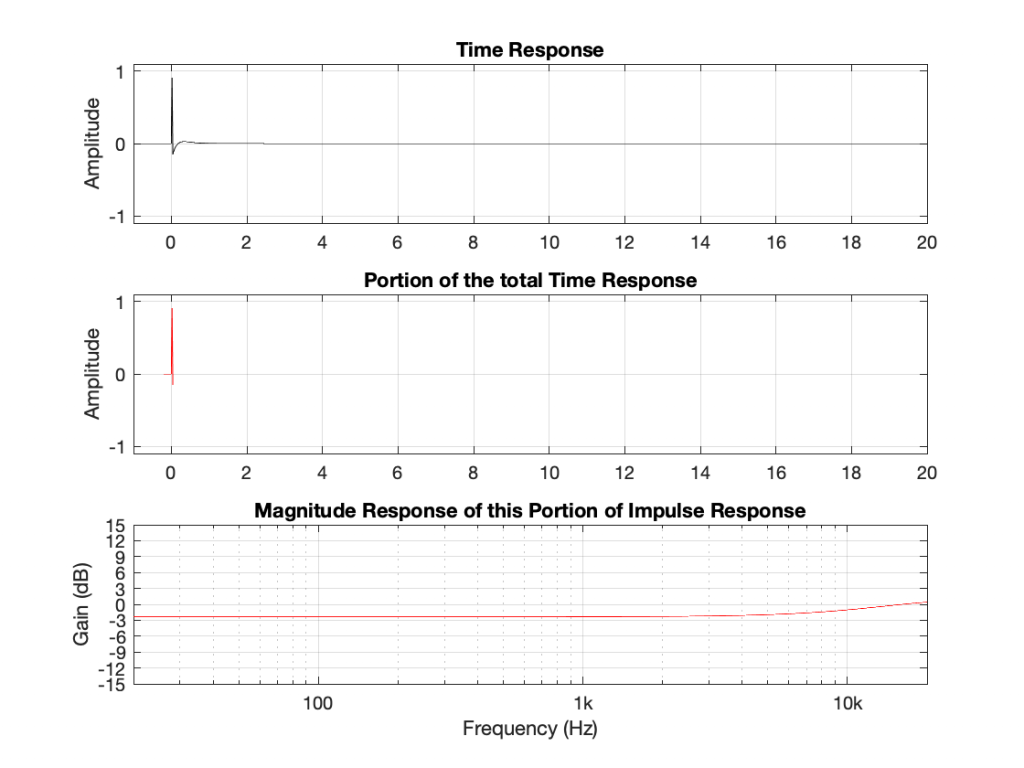Fig. 9: Similar to the boost, the first onset of the impulse has a nearly-flat magnitude response.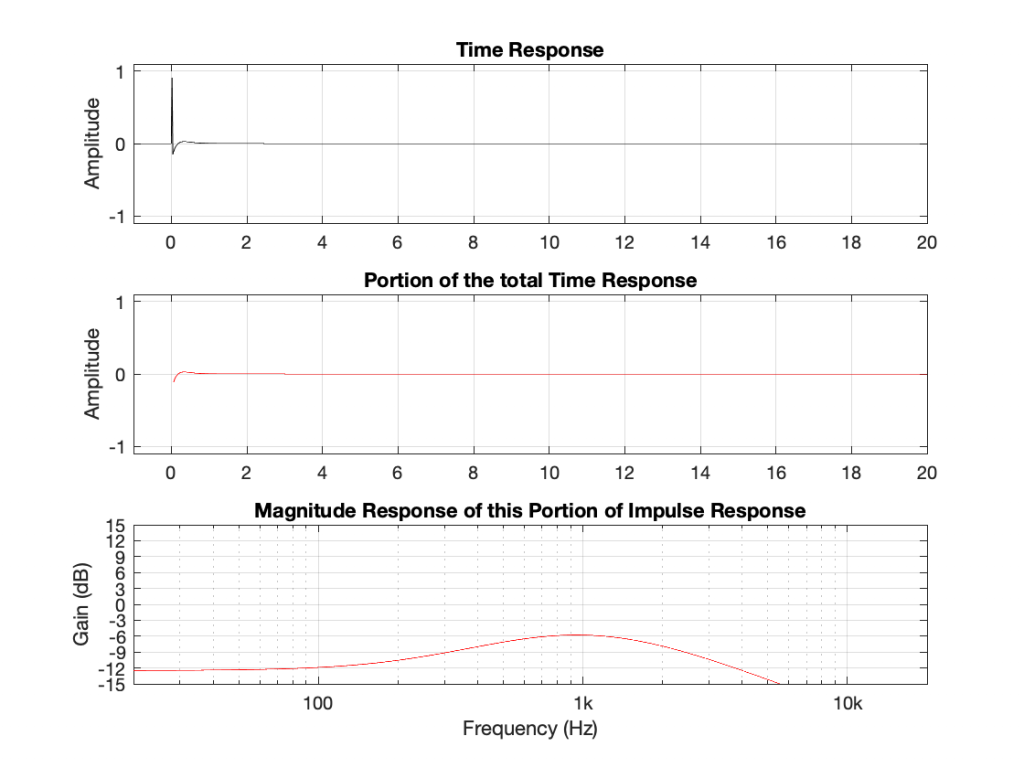Fig 10. The decay of the dip filter is also a slightly-strange-looking band-pass filter, but with an overall gain of about -6 dB.

Okay, what’s going on here? A peaking filter with a boost is a through-put plus a bandpass. A dip filter is ALSO a through-put plus a somewhat quieter (sort-of) bandpass. This doesn’t make any sense.

Actually it doesn’t make any sense because there’s a piece of information that I’m leaving out – the phase of the ringing. Notice that, with the peaking filter, the decay portion starts positive and then goes negative initially. With the dip filter, the decay starts negative and goes positive. So, the previous paragraph should have read: “A peaking filter with a boost is a through-put PLUS a bandpass. A dip filter is ALSO a through-put MINUS a somewhat quieter (sort-of) bandpass.”

The phases of the decays of the bandpass portions are opposite for the two filters. Another way to think of this is that the ringing in the dip filter cancels the energy around 1 kHz in the initial impulse, whereas the ringing in the peak filter adds to it.

However, it’s really important to note for now that both filters – the peak and the dip result in ringing in time.

# Filters and Ringing: Part 1

Let’s say that, for some reason, you want to apply an equaliser to an audio signal. It doesn’t matter why you want to do this: maybe you like more bass, maybe you need more treble, maybe you’re trying to reduce the audibility of a room mode. However, one thing that you should know is that, by changing the frequency response of the system, you are also changing its time response.

Now, before we go any farther, do NOT mis-interpret that last sentence to mean that a change in the time response is a bad thing. Maybe the thing you’re trying to fix already has an issue with its time response, and sometimes you have to fight fire with fire.

Before we start talking about filters, let’s talk about what “time response” means. I often work in an especially-built listening room that has acoustical treatments that are specifically designed and implemented to result in a very controlled acoustical behaviour. I often have visitors in there, and one of the things they do to “test the acoustics” is to clap their hands once – and then listen.

On the one hand (ha ha) this is a strange thing to do, because the room is not designed to make the sound of a single hand clap performed at the listening position sound “good” (whatever that means). On the other hand, the test is not completely useless. It’s a “play-toy” version of a very useful test we use to measure a loudspeaker called an impulse response measurement. The clap is an impulsive sound (a short, loud sound) and the question is “how does the thing you’re measuring (a room or a loudspeaker, for example) respond to that impulse?”

So, let’s start by talking about the two important reasons why we use an impulse.

## Time response

If a thing in a room makes a sound, then the sound radiates in all directions and starts meeting objects in its path – things like walls and furniture and you. When that happens, the surface it meets will absorb some amount of energy and reflect the rest, and this is balance of absorbed-to-reflected energy is different at different frequencies. A cat will absorb high frequencies and low frequencies will just pass by it. A large flat wall made of gypsum will reflect high frequencies and absorb whatever frequency it “wants” to vibrate at when you thump it with your fist.

The energy that is absorbed is (eventually) converted to heat: that’s lost. The reflected energy comes back into the room and heads towards another surface – which might be you as well, but probably isn’t unless you’re in a room about the size of an ancient structure known to archeologists as a “phone booth”.

At your location, you only hear the sound that reaches you. The first part of the sound that you hear “immediately” after the thing made the noise, probably travelled a path directly from the source to you. Let’s say that you’re in a large church or an aircraft hangar – the last sound that you hear as it decays to nothing might be 5 seconds (or more!) after the thing made the noise, which means that the sound travelled a total of 5 sec * 344 m/s = 1.72 km bouncing around the church before finally arriving at your position.

So, if I put a loudspeaker that radiates simultaneously in all directions equally at all frequencies (audio geeks call this a point source) somewhere in a room, and I put a microphone that is equally sensitive to all frequencies from all directions (audio geeks call this an omnidirectional microphone) and I send an impulse (a “click”) out of the loudspeaker and record the output of the microphone, I’ll see something like this:

Some things to notice about that plot shown above

• There is some silence before the first sound starts. This is the time it takes for the sound to get from the loudspeaker to the microphone (travelling at about 344 m/s, and with an onset of about 30 ms, this means that the microphone was about 10.3 m away.
• There are some significant spikes in the signal after the first one. These are nice, clean reflections off some surfaces like walls, the floor or the ceiling.
• Mostly, this is a big mess, so it’s difficult to point somewhere else and say something like “that is the reflection off the coffee mug on the table over there, after the sound has already hit the ceiling and two walls on the way” for example…

So, this shows us something about how the room responds to an impulse over time. The nice (theoretical) thing is that this is a plot of what will happen to everything that comes out of the loudspeaker, over time, when captured at the microphone’s position. In other words, if you know the instantaneous sound pressure at any given moment at the output of the point-source loudspeaker, then you can go through time, multiplying that value by each value, moment by moment, in that plot to predict what will come out of the microphone. But this means that the total output of the microphone is all of the sound that came out of the loudspeaker over the 1000 ms plotted there, with each moment individually multiplied by each point on the plot – and all added together.

This may sound complicated, but think of it as a more simple example: When you’re sitting and listening to someone speak in a church, you can hear what that person just said, in addition to the reverberation (reflections) of what they said seconds ago. There is one theory that this is how harmony was invented: choirs in churches noticed that the reverb from the previous note blended nicely with the current note, and so chords were born.

## Frequency Response

There is a second really good reason for using an impulse to test a system. An impulse (in theory) contains all frequencies at the same level. This is a little difficult to wrap ones head around (at least, it took me years to figure out why…) but let me try to explain.

Any sound is the combination of some number of different frequencies, each with some level and some time relationship. This means that, I can start with the “ingredients” and add them together to make the sound I want. If I start with two frequencies: 1 Hz and 2 Hz and add them together, using cosine waves (a cosine wave is the same as a sine wave that starts 90º late), the result is as shown in Figure 2.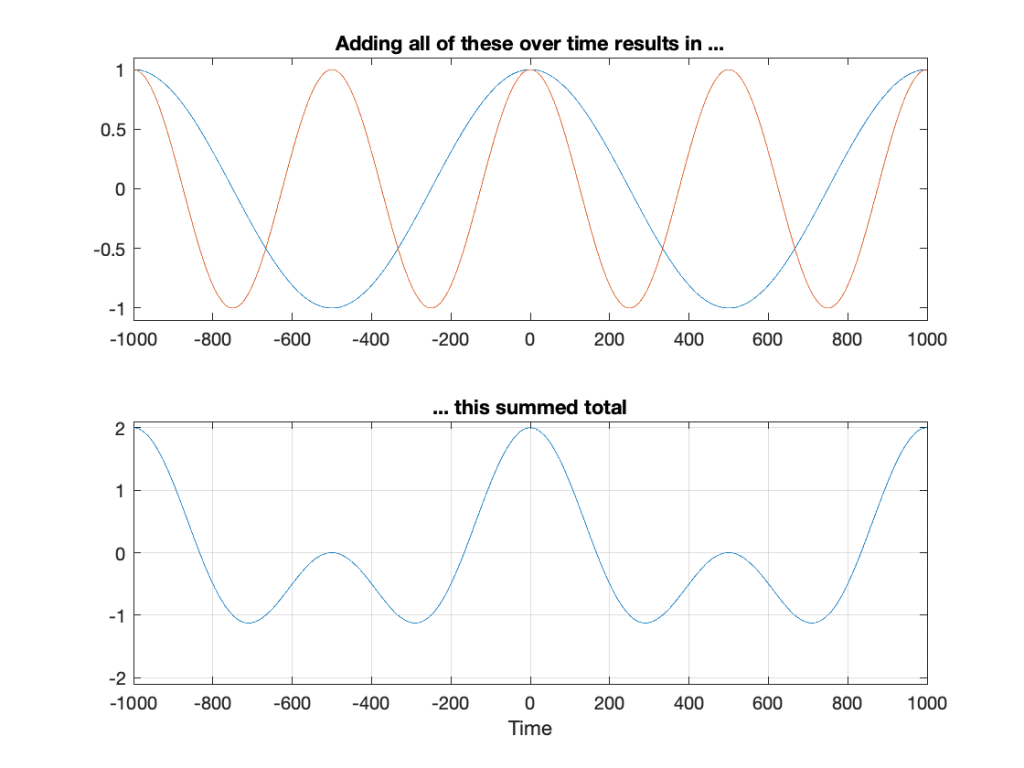Fig 2. The top plot shows two cosine waves with frequencies of 1 Hz (blue) and 2 Hz (red). The bottom plot is the result of adding them together, point by point, over time. For example, at Time = 0 ms, you can see the result is 1+1 = 2. At Time = 500 ms, the result is 1 + -1 = 0.

Let’s do this again, but increase the number to 5 frequencies: 1 Hz, 2 Hz, 3 Hz, 4 Hz, and 5 Hz.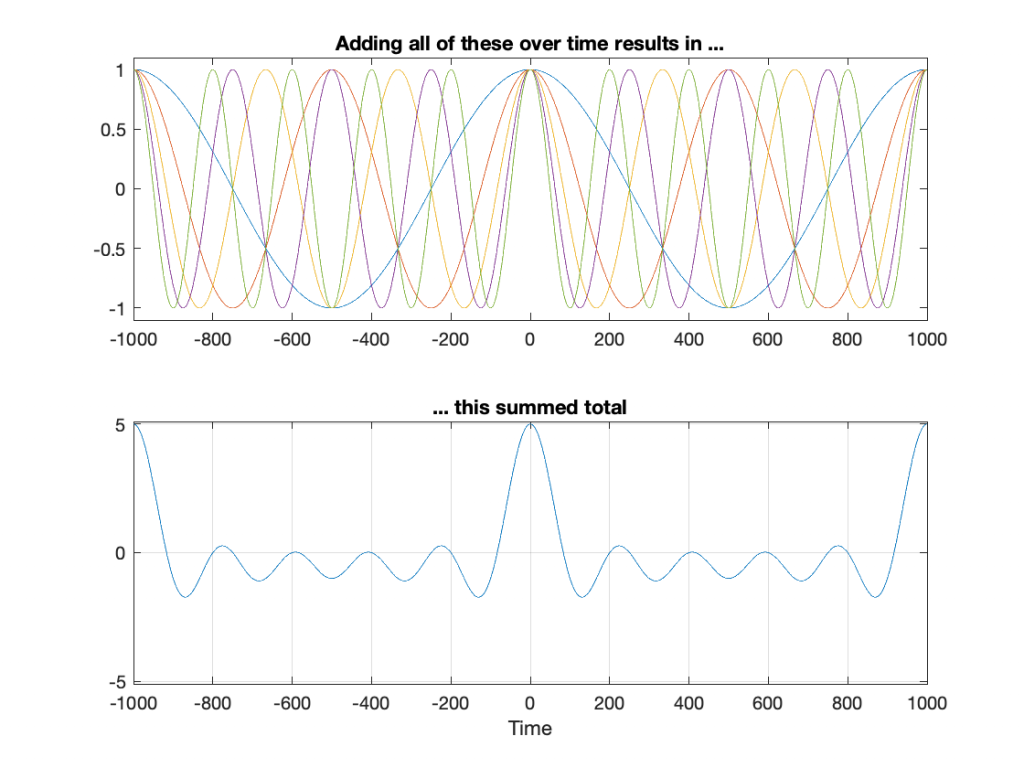Fig 3. Adding 5 frequencies results in a different total – notice, though that the peak at Time = 0 ms is 5, for example.

You may notice that the peak at Time = 0 ms is getting bigger relative to the rest of the result. However, we get the same peak values at Time = -1000 ms and Time = 1000 ms. This is because the frequencies I’m choosing are integer values: 1 Hz, 2 Hz, 3 Hz, and so on. What happens if we use frequencies in between? Say, 0.1 Hz to 10 Hz in steps of 0.1 Hz, thus making 100 cosine waves added together? Now they won’t line up nicely every second, so the result looks like Figure 4.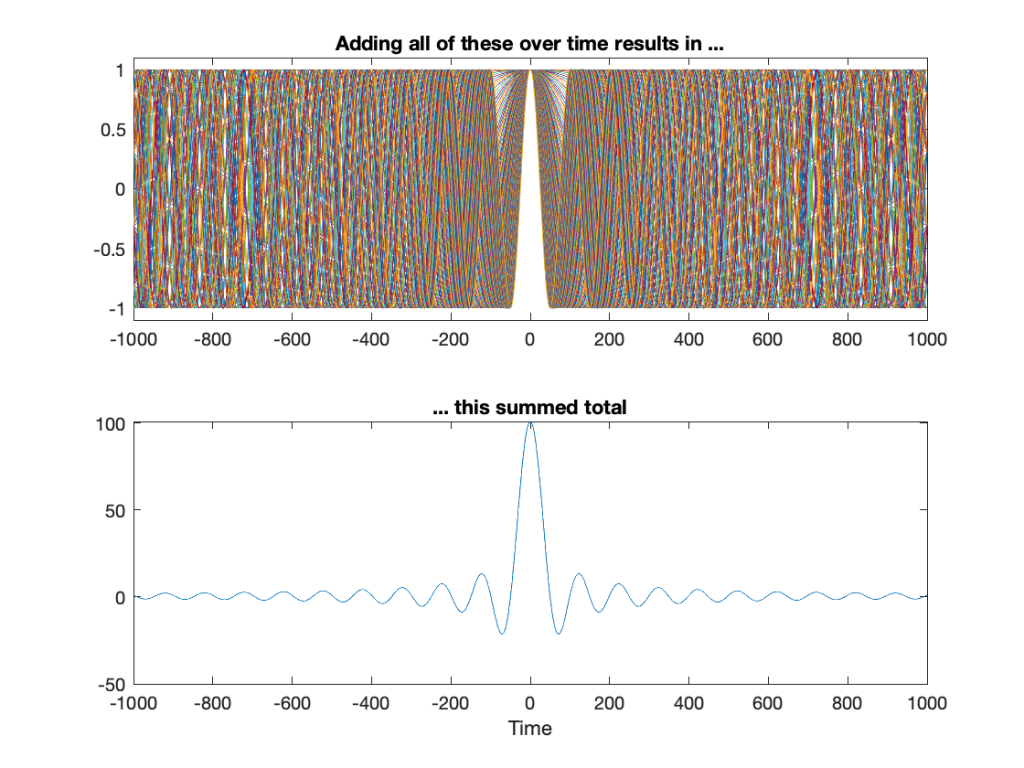Fig 4. Adding 100 frequencies from 0 Hz to 10 Hz in steps of 0.1 Hz looks ugly at the top because of all of the overlapping plots. However, those overlapping plots start to cancel each other out, so we get a big peak where they all hit 1 (at Time = 0 ms) and approach 0 at all other times.

Let’s get crazy. Figure 5 shows 10,000 cosine waves with frequencies of 0 to 100 Hz in steps of 0.01 Hz.

You may start to notice that the result of adding more and more cosine waves together at different frequencies is starting to look a lot like an impulse. It’s really loud at Time = 0 ms (whenever that is, but typically we think that it’s “now”) and it’s really quiet forever, both in the past and the future.

So, the moral of the story here is that if you click your fingers and make a “perfect” impulse, one philosophical way to think of this is that, at the beginning of time, cosine waves, all of them at different frequencies, started sounding – all of them cancelling each other until that moment when you decided to snap your fingers at Time = 0. Then they all continue until the end of time, cancelling each other out forever…

Or, another way to think of it is simply to say “an impulse contains all frequencies, each with the same amplitude”.

One small point: you may have noticed in Figure 5 that the impulse is getting big. That one added up to 10,001 – and we were just getting started. Theoretically, a real impulse is infinitely short and infinitely loud. However, you don’t want to make that sound because an infinitely loud sound will explode the universe, and that will wreck your analysis… It will at least clip your input.

## Equalisation

Let’s take a simple example of an equaliser. I’ll use an EQ to apply a boost of 12 dB with a centre frequency of 1 kHz and a Q of 2. (Note that “Q” has different definitions. The one I’ll be using here is where the Q = Fc / BW, where BW is the bandwidth in Hz between the -3 dB points relative to the highest magnitude. If you want to dig deeper into this topic, you can start here.) That filter will have a magnitude response that looks like this: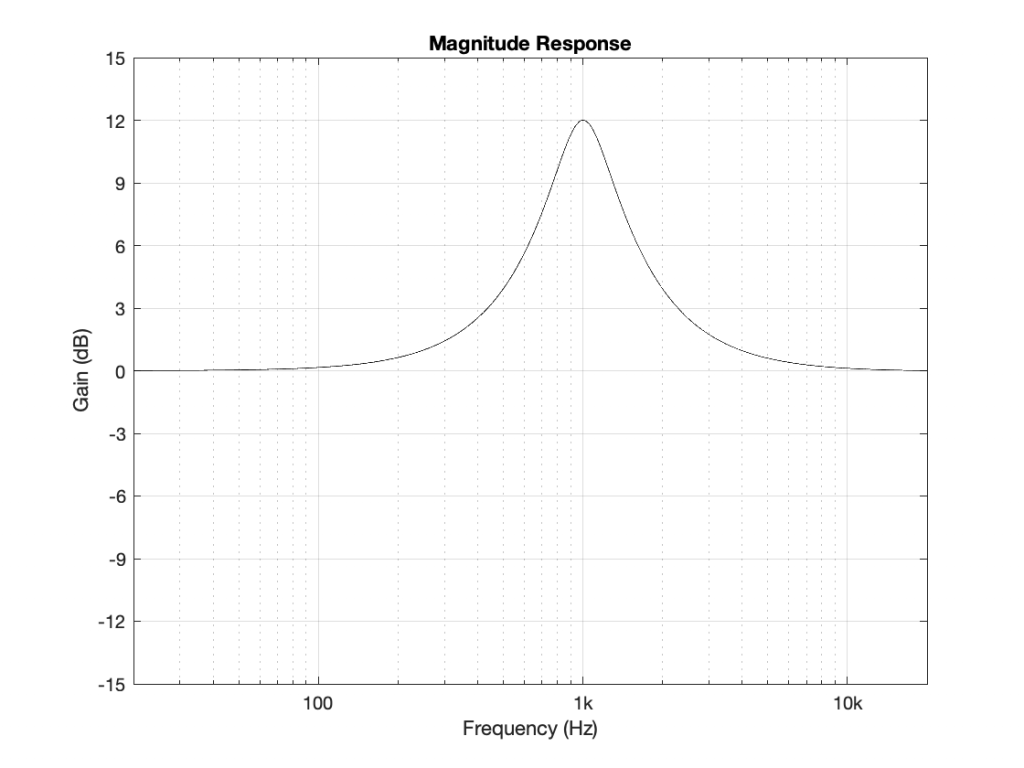Fig 6. The gain response of an equaliser using a peaking filter where Fc = 1 kHz, Gain = +12 dB, and Q = 2.

As you can see there, this means that a signal coming into that filter at 20 Hz or 20 kHz will come out at almost exactly the same level. At 1000 Hz, you’ll get 12 dB more at the output than the input. Other frequencies will have other results.

The question is: “how does the filter do that, conceptually speaking?”

That’s what we’ll look at in the next part of this series.

# Heavy Metal Analogue

In order to explain the significance of the following story, some prequels are required.

Prequel #1: I’m one of those people who enjoys an addiction to collecting what other people call “junk” – things you find in flea markets, estate sales, and the like. Normally I only come home with old fountain pens that need to be restored, however, occasionally, I stumble across other things.

Prequel #2: Many people have vinyl records lying around, but not many people know how they’re made. The LP that you put on your turntable was pressed from a glob of molten polyvinyl-chloride (PVC), pressed between two circular metal plates called “stampers” that had ridges in them instead of grooves. Easy of those stampers was made by depositing layers of (probably) nickel on another plate called a “metal mother” which is essentially a metal version of your LP. That metal mother was made by putting layers on a “metal master” (also with ridges instead of grooves) which was probably a lamination of tin, silver, and nickel that was deposited in layers on an acetate lacquer disc, which is the original, cut on a lathe. (Yes, there are variations on this process, I know…) The thing to remember in this process is

• there are three “playable” versions of the disc in this manufacturing process: your LP, the metal mother, and the original acetate that was cut on the lathe
• there are two other non-playable versions that are the mirror images of the disc: the metal master and the stamper(s).

(If you’d like to watch this process, check out this video.)

Prequel #3: One of my recurring tasks in my day-job at Bang & Olufsen is to do the final measurements and approvals for the Beogram 4000c turntables. These are individually restored by hand. It’s not a production-line – it really is a restoration process. Each turntable has different issues that need to be addressed and fixed. The measurements that I do include:

• verification of the gain and response of the two channels in the newly-built RIAA preamplifier
(this is done electrically, by connecting the output of my sound card into the input of the RIAA instead of using a signal from the pickup)
• checking the sensitivity and response of the two channels from vinyl to output
• checking the wow and flutter of the drive mechanism
• checking the channel crosstalk as well as the rumble

The last three of these are done by playing specific test tracks off an LP with signals on it, specifically designed for this purpose. There are sine wave sweeps, sine waves at different signal levels, a long-term sine wave at a high-ish frequency (for W&F measurements), and tracks with silence. (In addition, each turntable is actually tested twice for Wow and Flutter, since I test the platter and bearing before it’s assembled in the turntable itself…)

Prequel #4: Once-upon-a-time, Bang & Olufsen made their own pickup cartridges (actually, it goes back to steel needles). Initially the SP series, and then the MMC series of cartridges. Those were made in the same building that I work in every day – about 50 m from where I’m sitting right now. B&O doesn’t make the cartridges any more – but back when they did, each one was tested using a special LP with those same test tracks that I mentioned above. In fact, the album that they used once-upon-a-time is the same album that I use today for testing the Beogram 4000c. The analysis equipment has changed (I wrote my own Matlab code to do this rather than to dust off the old B&K measurement gear and the B&O Wow and Flutter meter…)

If you’ve read those four pieces of information, you’ll understand why I was recently excited to stumble across a stamper of the Bang & Olufsen test LP, with a date on the sleeve reading 21 March, 1974. It’s funny that, although the sleeve only says that it’s a Bang & Olufsen disc, I recognise it because of the pattern in the grooves (which should give you an indication of how many times I’ve tested the turntables) – even if they’re the mirror image of the vinyl disc.

Below, you can see my latest treasure, pictured with an example of the B&O test disc that I use. It hasn’t “come home” – but at least it’s moved in next-door.

• No, the test disc is no longer available – it never was outside of the B&O production area. However, if you can find a copy of the Brüel and Kjær QR 2010 disc, it’s exactly the same. I suspect that the two companies got together to produce the test disc in the 70s. However, there were also some publicly-available discs by B&O that included some test tones. These weren’t as comprehensive as the “real” test discs like the ones accompanying the DIN standards, or the ones from CBS and JVC.
• No, the metal master is no longer in good enough shape to use to make a new set of metal mothers and stampers. Too bad… :-(

P.P.S. If you’re interested in the details of how the tests are done on the Beogram 4000c turntables, I’ve explained it in the Technical Sound Guide, which can be downloaded using the link at the bottom of this page. That document also has a comprehensive reading list if you’re REALLY interested or REALLY having trouble sleeping.

# Fixed point vs. Floating Point

When an analogue audio signal is converted to a digital representation, the value of the level for each sample is rounded to the nearest quantisation step (because a digital audio system does not have an infinite resolution). I’ve talked about this in detail in a past posting.

When a sample value in a digital audio stream is stored or transmitted inside a piece of audio equipment or software, one of the choices the engineer can make is whether the value should be represented using a fixed point or a floating point system. These are related, but fundamentally different, and they have some effects on the audio signal that may be audible if you’re not careful…

Let’s lay down some basic points to start. We’ll say the following:

• Audio is a kind of AC signal that has a level that can vary between two values.
• For now, we’ll say that the limits on the range of values is -1 and +1, and it can be anything in between.
• We’re going to divide up that range into some finite number of steps and round the actual signal value to the closest usable value. (I’ll assume for this posting that you already understand that dither is your friend.)
• The value will be stored as a binary number somehow

The question that we’ll look at here is exactly how that binary value represents the number, and a little of what that means to the audio signal.

## Fixed Point Representation

The simplest way to represent the value is to divide the total range from the minimum to the maximum number into an equal number of steps, and round the signal’s value to the closest step. This is a really generalised description of a “fixed point” system.

For example, if we have a 3-bit number to play with, we’ll take the first bit and use that one to represent the + or – portion of the value (where 0 means “+” and 1 means “-“). For values from 0 up to (just under) the positive maximum, the other 2 bits are used to just count the steps, from 000 up to 011. The negative values start at the bottom and work their way up to 1 step below 0, from 100 to 111. This can be seen in Figure 1.Figure 1: A simplified representation of the use of quantisation steps in a 3-bit fixed point system.

If you look carefully at Figure 1, you’ll see that there is one extra negative step, since one of the positive steps is used to represent the value 0 in the middle. This means that, if the signal is symmetrical, then we will wind up using all of the possible quantisation values except for the bottom one (just like I’ve shown in the plot), however, for the rest of this discussion, we’ll be working with numbers that are so big that this one step doesn’t really matter, so I won’t mention it again.

If we are using a 3-bit number to represent the value, then we have a total number of 23 quantisation steps: 8 of them. Each time we add one more bit, we double the number of steps. So, for a 16-bit sample, we have 216, or 65,536 possible quantisation values. For a 24-bit sample, we have 224, or 16,777,216 steps.

By increasing the number of bits in the number, we don’t change the level (it still has a range of -1 to +1), we’re just increasing the resolution that we have to make the measurement. The higher the resolution, the lower the error, and so the lower the level of distortion (if we don’t dither) or noise (if we do) relative to the signal.

If you have a fixed-point system, and you want to calculate the difference in level between the maximum signal level and the noise floor, then you can use a somewhat simplified equation, shown below:

Dynamic Range In dB ≈ 6 * nBits – 3

As I said, this is simplified due to some rounding to keep the numbers nice, but the general idea is that you have a doubling of dynamic range for every extra bit (therefore 6 dB per bit) and you lose 3 dB for the (TPDF) dither (but that’s better than not having the dither and having distortion instead). If you wanted to do it properly, then you can use this math instead:

Dynamic Range In dB ≈ 20*log10(2nBits) – 20*log10(sqrt(2))

So, if you have a 16-bit fixed point system, you have about 93 dB of range from the loudest signal to the noise floor. If you have a 24-bit system, it’s about 141 dB.

Remember that the noise floor is constant (I’m assuming it’s dithered), so as the signal level drops below maximum the current signal to noise ratio will drop by the same amount. Therefore, if your signal is 12 dB below maximum (or -12 dB FS, which means “12 decibels below Full Scale”), then the SNR in a 16-bit system is 93 – 12 = 81 dB.

If that last paragraph didn’t make complete sense, go back and read it again, because it’ll come back later…

Fixed point is a good system for conversion of an audio signal from and to analogue, but if you’re doing some really serious processing, it might not work out so well. This is due to two primary reasons:

• If your signal is going to outside the range, it will clip at the maximum positive or the minimum negative value because fixed point is not designed to exceed its range.
• If the signal is going to be reduced to a very low level somewhere in your proceeding (say, inside a biquad, for example) then you might need a LOT of bits to keep the noise floor low enough when the signal level is brought back up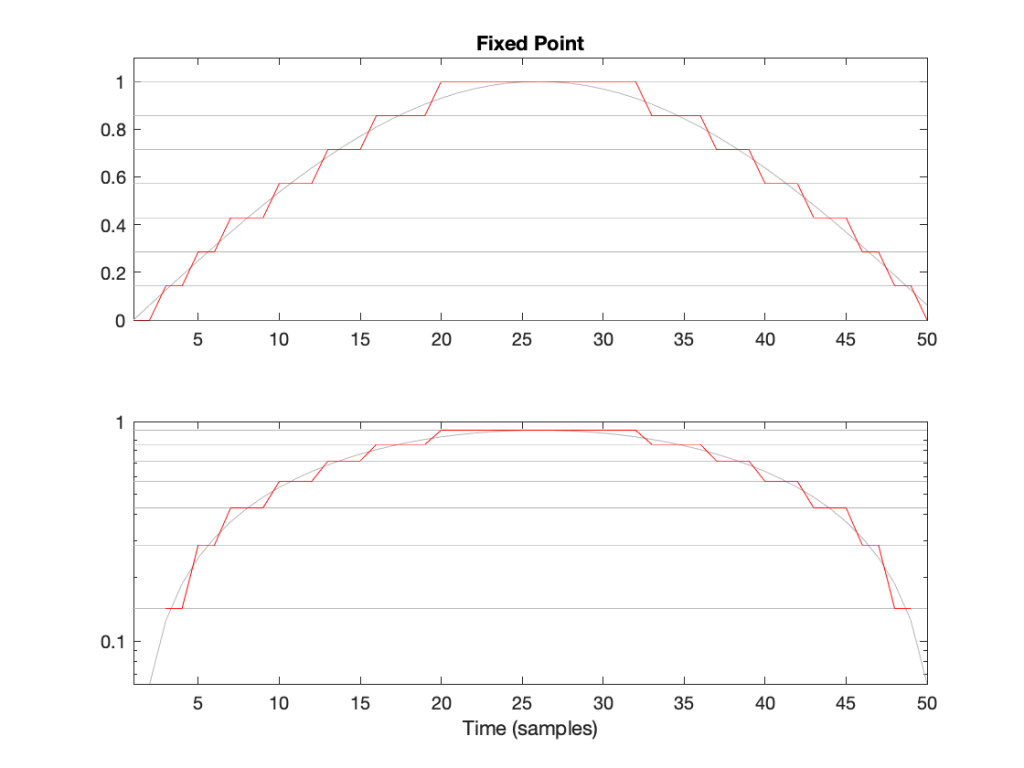Figure 2: The first half of a sine wave (in grey) quantised (without dither) in a simplified 4-bit fixed point system. (I’ve actually cheated a bit and just made 8 equally-spaced steps from 0 to 1 unlike the version shown in Figure 1.) The two plots show identical data, but the bottom plot has a logarithmically-scaled Y-axis.

As can be seen in Figure 2, the equally-spaced steps in a fixed point world mean that the quantisation error is always between -0.5 and 0.5 of a step (a “Least Significant Bit” or LSB), regardless of the level of the signal.

## Floating Point Representation

There is another way to use the bits to represent the signal value. This is to divide the binary “word” into two parts and to do a little math involving some subtraction, multiplication, and an exponent to arrive at the value. Just like in the Fixed Point case, we’ll reserve one bit for the +/- indicator.

Let’s say that we have a 32-bit value to work with. We’ll divide this up into the following:

• 23 bits for the fraction or mantissa, which we’ll abbreviate f
• 8 bits for the exponent, abbreviated e
• 1 bit for the +/- sign (just like in Fixed Point)

We’ll then do the following math:

Sample Value = ± (1 – f) * 2e

We need to know a little extra information:

• because we’re using 23 bits for f, then it can range from 0 to 223-1. In other words, stated mathematically:
0 ≤ 223*f < 223
• because we’re using 8 bits for e, then it has a total range of 28 possible values. In other words it has a range from just over -27 to just under 27. In other words, stated mathematically:
-126 ≤ e ≤ 127
(Note that a couple of possible values are reserved for special purposes, but we won’t talk about those)

This is all a little complicated, but there is a “punch line” to which I’m headed:

Unlike Fixed Point representation, the divisions of the values – the number of steps, and therefore the step sizes – are not the same across the entire scale of possible values. It’s divided into sections, where each section has quantisation steps of equal size, but that step size is dependent on what the value is. In other words the step size changes with the value, but on a coarser scale.

That step size can be calculated as follows:

From 2e to 2e+1, the steps all have an equal size of 2e-fBits where fBits is the number of bits used to express f (in the case of a 32-bit floating point word, fBits = 23 bits). In other words, we have 2fBits equally-spaced steps in that range.

Therefore, each time the signal value moves from just below 0.5 to just above (for example) then the resolution changes, and the higher the value, the lower the resolution. This is is how Floating Point representation behaves.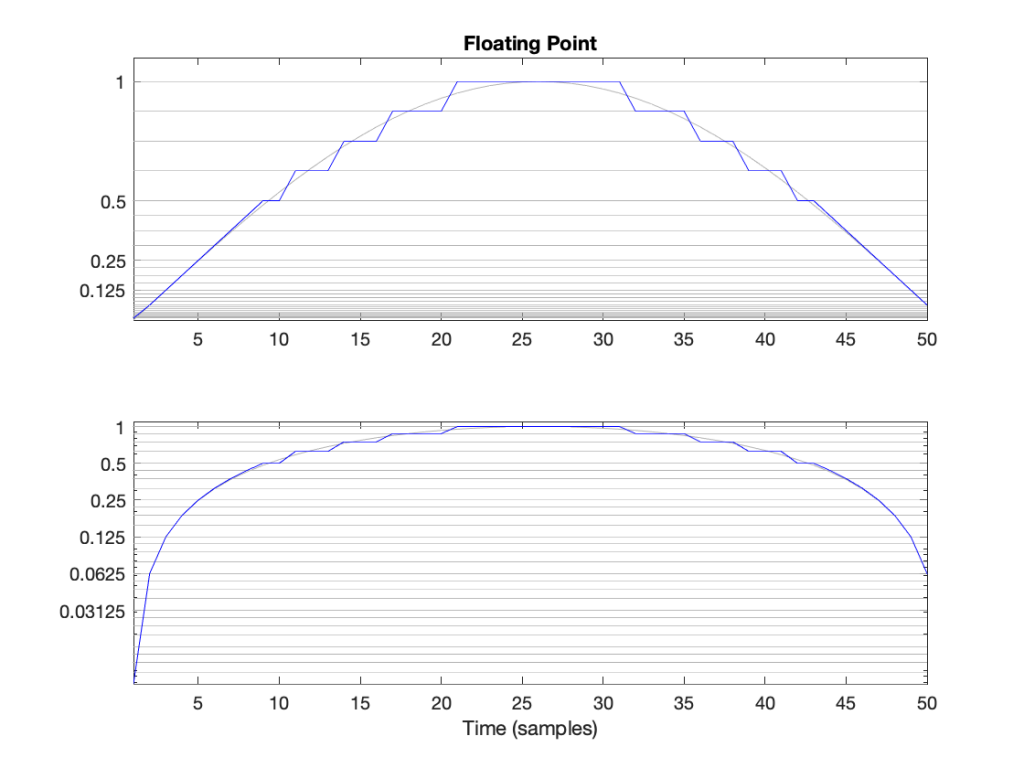Figure 3: The first half of a sine wave (in grey) quantised (without dither) in a simplified floating point system with 2 bits for the fraction. This means that there are 4 equally-spaced steps from (for example) 0.25 to 0.5 or 0.5 to 1. The two plots show identical data, but the top plot has a linearly-scaled Y-axis, whereas the bottom plot has a logarithmically-scaled Y-axis.

## Do I care?

Let’s find out.

In a 32-bit floating point world (therefore, one with a 23-bit fraction), if I have a signal that has a level that has has a maximum positive value of 1 (or 20), then the resolution of the value (which defines the error, which defines the “distance” in dB to the noise floor) is 2-25 (or 1/33,554,432).* This means that the noise floor is about 150 dB below the signal (20 * log10(1 / 2-25). As the signal level drops to 0.5, the noise floor remains the same, so the signal drops by 6 dB, and the SNR reduces to 150 – 6 = 144 dB.

Then, when we drop just below 0.5, the resolution of the value suddenly changes to 2-26 (or 1/67,108,864) , which means that the noise floor is about 150 dB below the signal (20 * log10(0.5 / 2-26). As the signal drops to 0.25 (-6 dB relative to 0.5), the noise floor remains the same, so the signal drops by 6 dB, and the SNR reduces to 150 – 6 = 144 dB.

Then, when we drop just below 0.25, the resolution of the value suddenly changes to 2-27 (or 1/134,217,728), which means that the noise floor is about 150 dB below the signal (20 * log10(0.25 / 2-27). As the signal drops to 0.25 (-6 dB relative to 0.5), the noise floor remains the same, so the signal drops by 6 dB, and the SNR reduces to 150 – 6 = 144 dB.

Hopefully, by now, you’re seeing a pattern here.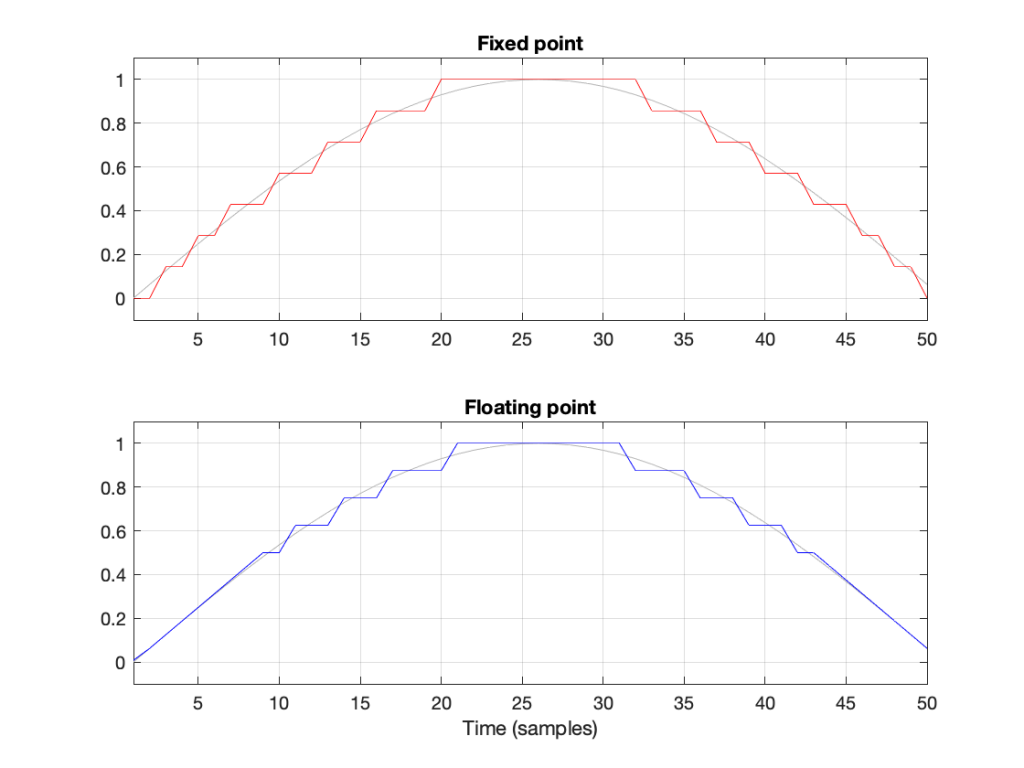Figure 4: Notice that the error of the floating point version is reduced when the signal level (in grey) approaches 0.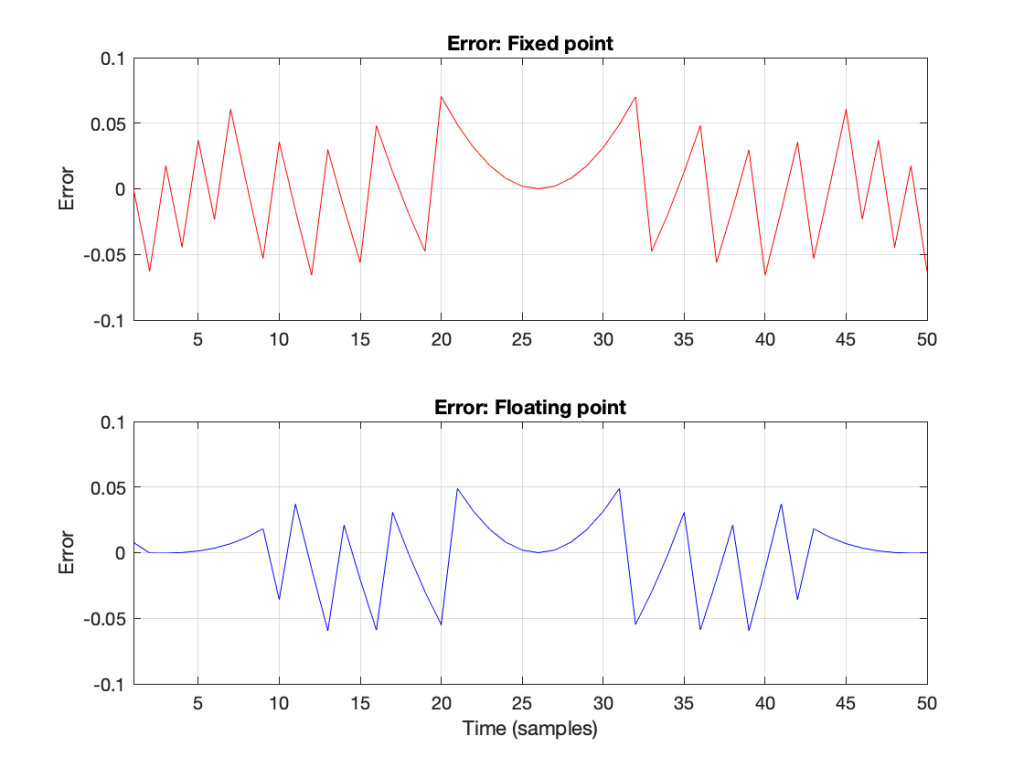Figure 6: The errors from the quantisation shown Figure 5. These are just the original signal subtracted from the quantised signals. Notice that, in Floating Point, the general level of the error is dependent on the level of the signal (it’s smaller on the left and right of the plot) whereas in Fixed Point, the overall level of the error is more constant.

The cool thing is that the pattern would have been the same if I had gone above 1 instead of below it. So, the two things to worry about in Fixed Point (inadequate resolution with (temporarily) low-level signals and clipping when the signal goes outside the range) are not problems in floating point.** And, if you have enough bits (32-bit floating point is the standard “single precision” resolution, but 64-bit “double precision” resolution is not uncommon).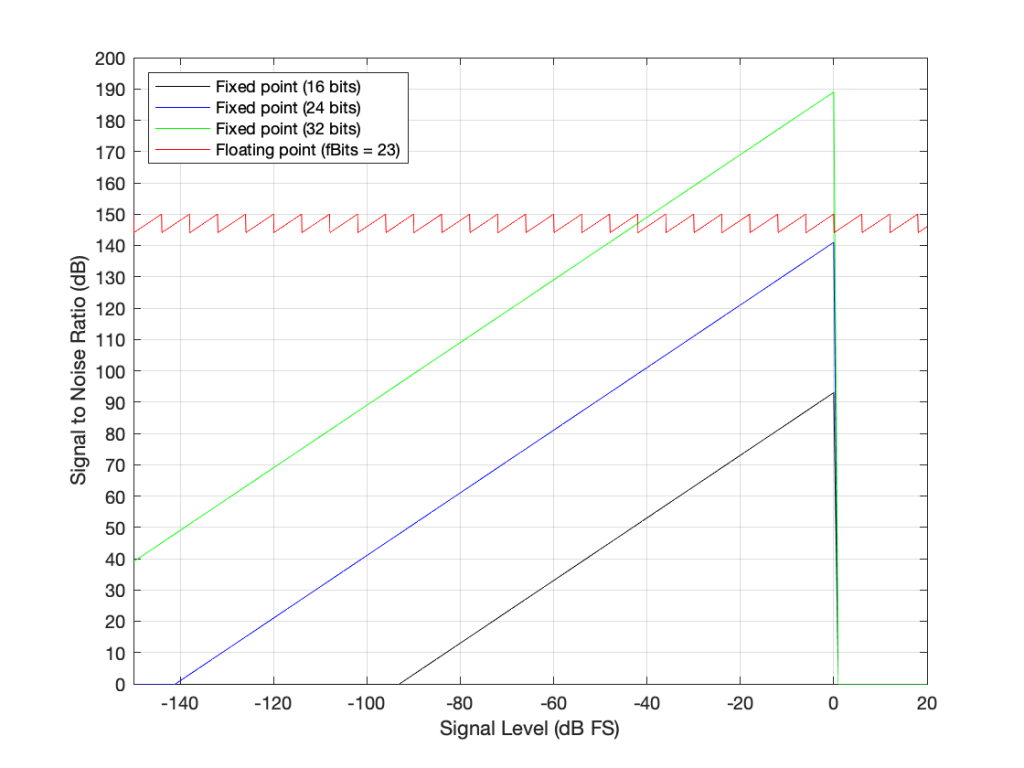Figure 7: The Signal to Distortion+Noise ratio of four different systems, as a function of the signal level in dB FS.**

This is why, in most modern audio systems, you have a fixed-point ADC and a DAC (an Analogue to Digital Converter and a Digital to Analogue converter) at the input and output of your system (because the signal range is reasonably well-defined, and the dynamic range is more than adequate if you do it right) but the processing on the inside is done in 32-bit or 64-bit floating point (or both, in some devices) so that the engineers have the resolution and the range to play with the signals before getting them ready for the output.***

There may be some argument made for a constant noise floor level in a fixed-point system (assuming it’s dithered) over a signal-modulated noise level in a floating-point world (assuming it’s not), however, there are two reasons why this is likely not a real-world issue. The first is that, even in a single-precision floating point system, the worst-case signal to noise ratio is about 144 dB, which is very good. The second is that smart people have already been thinking about dither for floating point systems. If this sounds interesting, you can start reading here

## One last thing

You may be wondering about that sawtooth plot: the red line in Figure 7. It can’t keep going forever, right?

Right.

Eventually, if the signal is quiet enough, then you run out of exponents and the system just behaves as a 23-bit fixed point system (assuming a 32-bit floating point). This will happen when e = -126. Below that, then the SNR just follows a downward slope just like the fixed-point plots. If the signal is loud enough (when e = 127) then you’ll clip, again, just like the fixed-point systems do when the input signal has a level of 0 dB FS.

So, then the question is: “how quiet / loud does the input signal have to be for that to happen?” The answer is very quiet and very loud, as you can see in the plot in Figure 8.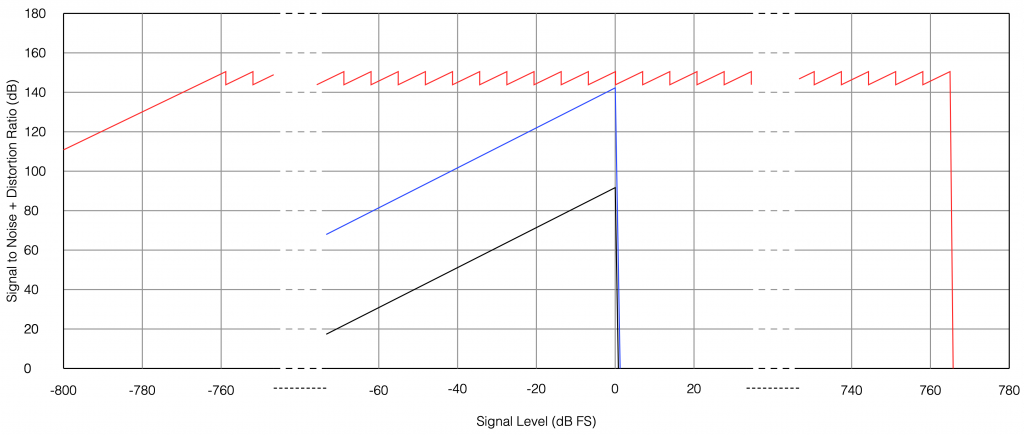Fig 8. The limits of a 32-bit floating point signal. As you can see, you’ve got plenty of dynamic range to work with before you run out of room on either side. The black line is 16-bit fixed point, the blue line is 24-bit fixed point, and the red line is 32-bit floating-point.

You may be wondering how I calculated those limits:

• The first peak in the sawtooth on the left side is at 20*log10(2^-126) = -758.6 dB FS
• The last peak in the sawtooth on the right side is at 20*log10(2^127) = 764.6 dB FS
• The slope that just below the 0 dB FS Signal level is where e = -1. The slope just above 0 dB FS is where e = 0.

## * First small note for the attentive

You may have noticed what appears to be a mistake in my math in there. First I said:

From 2e to 2e+1, the steps all have an equal size of 2e-fBits where fBits is the number of bits used to express f (in our case, fBits = 23 bits). In other words, we have 2fBits equally-spaced steps in that range.

Then I did the math and said

In a 32-bit floating point world (therefore, one with a 23-bit fraction), if I have a signal that has level that has just come up to 1 (or 20), then the resolution of the value (which defines the error, which defines the “distance” in dB to the noise floor) is 2-25 (or 1/133,554,432).

Why did I say 2-25 when maybe I should have said 2-23 (because there are 23 bits in the fraction)? The reason is that the 223 quantisation levels are located between 1 down to 0.5. If I were to continue with the same spacing down to 0, then I would have twice as many quantisation levels, so there would be 224 instead. If I were to continue the spacing all the way down to -1, then there would be twice as many again, or 225.

In other words, a floating point signal ranging from a value of 2-1 to 20 (0.5 to 1) with some number of bits in the fraction that we’re calling fBits will have almost exactly the same signal to noise ratio as an non-dithered fixed point system that is scaled to range from -1 to 1 with fBits+2.

This would be the same from -20 to -2-1 (-1 to -0.5).

At any other signal value, the quantisation behaviours (and therefore the signal-to-noise ratios) of the two systems will be significantly different.

This is visible in Figure 6 where, when the signal is high (in the middle of the plots), the error level is approximately the same in the 4-bit fixed-point system and the floating point system with 2 bits for the fraction.

## ** Second small note for the attentive

You will notice that the black, blue, and green lines in Figure 7 have a sharp transition when the signal level hits 0 dB FS. This is because, in a fixed point system at signal levels below 0 dB FS, the signal to noise ratio is the difference in level between the dither’s noise floor and the signal. The dither level is constant, so as the signal level increases, it gets “further away” from the noise floor until you reach 0 dB FS (with a sine wave), as which point you reach the maximum possible SNR. However, once the signal goes beyond 0 dB FS (still assuming it’s a sine wave), then it starts to clip and distortion components are generated. It does not take much increase in level to drastically increase the level of the distortion relative to the level of the signal (since the signal level cannot increase – you’re just increasing distortion artefacts). Consequently, the signal to distortion+noise drops dramatically, because the distortion components increase in level dramatically.

This does not happen with the floating point system because, at 0 dB FS, you just change the exponent and keep going up with the signal level until you reach the maximum possible exponent value, which goes far beyond what I’ve plotted here.

## Third small note for the attentive

You may be looking at Figure 7 and wondering why the fixed point plots and the floating point plots don’t overlap anywhere. For example, look where the green line (32-bit fixed point) crosses the red line (32-bit floating point). Why don’t they overlap each other there for that little 6 dB-wide range on the X-axis?

The reason is that I’m modelling the fixed point SNRs with TPDF dither, which “costs” 3 dB, but I’m assuming that the floating point signal is not dithered (which would normally be the case). If I were pretending that fixed point didn’t include the dither, then the plots would, indeed, overlap each other for that narrow little window.

## ***One last comment

You may be saying to yourself “But this is nonsense! Why do I need 150 dB SNR when the signal level is lower than -100 dB FS?” The long answer is in this posting, but the short answer is that the signal can go VERY low and VERY high inside a filter (a biquad), so you need to worry about this if you’re doing any changes to the magnitude response of the signal, for example…

Floating Point Numbers posted by Cleve Moler at Mathworks

Floating Point Denormals, Insignificant But Controversial posted by Cleve Moler at Mathworks

# Quantisation of Poles in the Z-plane

Back in this posting, I talked about biquads and their use in digital signal processing for making linear filters (what most of us call “equalisers”). Part of that explanation showed that a biquad is formed of a feed-forward section and a feed-back section, and you could swap the order of these two and get the same results. In a “Direct Form 1” implementation, the feed-forward comes first, as shown in Figure 1.

in the “Direct Form 2” implementation, the feed-back comes first, as shown in Figure 2.

There are advantages and disadvantages to each of these implementations, depending on things like how the rest of your system is implemented, and what, exactly you’re expecting the biquad to do.

However, for this posting, we’re going to “zoom in” a bit to the feed-back portion of the above diagrams. This portion of the biquad provides the “poles” in the Z-plane, as I described in the “Intuitive Z-plane” series of postings last week.

If I separate the feed-back portion of the above figures, it would look like Figure 3:

The locations of the poles (and therefore the magnitude response) of this portion of the filter are dependent on the gains at the outputs of the two 1-sample delays. What happens when these gains do not have an infinite resolution (which they can’t, because everything in a digitally-represented world is quantised to a finite number of steps)?

Before we go any further with this, I have to put in a reminder that quantisation (or “rounding”) in a DSP world is done in binary – not decimal. So, the quantisation that I’m about to do isn’t the same as stopping a couple of digits after the decimal…

In a world with infinite resolution, I can set the values of those two gains to be anything I want, and therefore the poles in my system can be anywhere within the circle. (We’ll assume that we don’t want a pole on the circle because that would result in a gain of ∞ dB, which is very loud.) However, let’s say that we quantise the gain values to a 4-bit binary value, where the first bit is reserved for the +/- indicator. This means that we only have +/- 2^3 possibilities, or 8 values, one of which is +0, and another is -0 (which is the same thing…). So, in other words, we have 7 possible negative values, 7 possible positive values, and 0.

The end result of this is that the poles have a limited number of locations where they can be placed. For the 4-bit quantisation described above, the resulting locations look like Figure 4.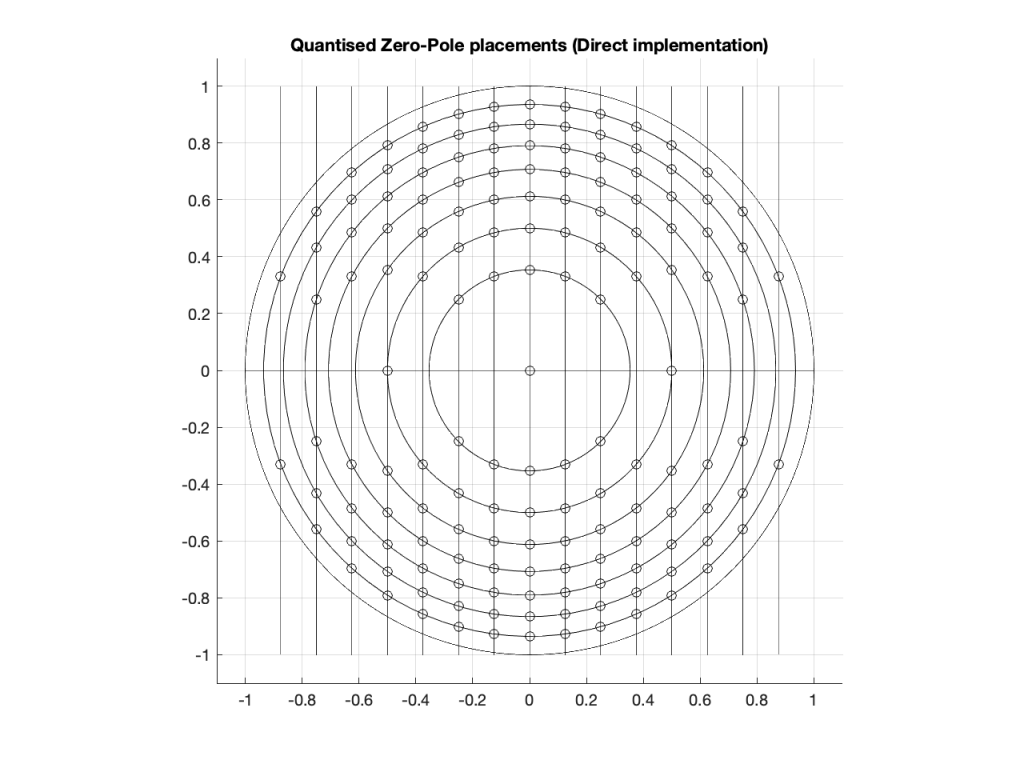Figure 4: The circles indicate the possible locations of the poles as a result of quantisation of the gain coefficients in a 4-bit Direct Form implementation.

Remember that we’re not talking about quantisation of the audio signal – it’s quantisation of the gain coefficients inside the biquad that will have an impact on the response of the filter.

Of course, it would be crazy to implement a biquad using only 4-bit quantisation for the gain coefficients. However, the point of this posting is not to show that biquads suck. It’s only to show one possibly important aspect of them if you’re a DSP engineer – but we’re getting there.

Just for fun, let’s increase the resolution of the system to 6 bits: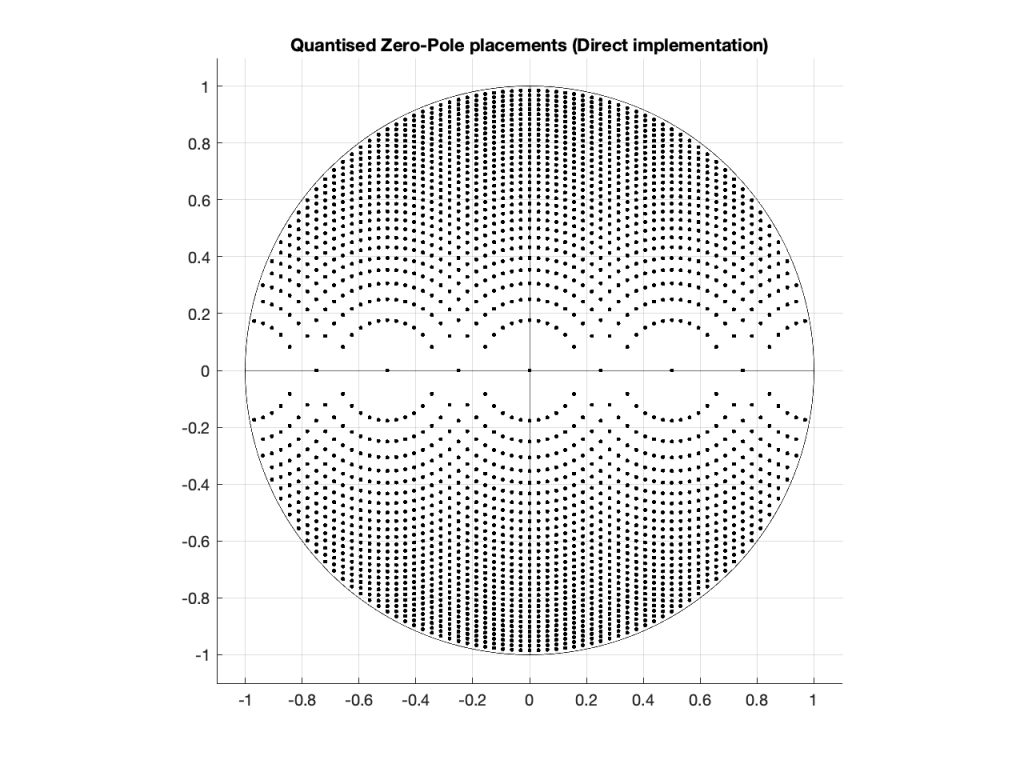Figure 5: The dots indicate the possible locations of the poles as a result of quantisation of the gain coefficients in a 6-bit Direct Form implementation.

… or to 8 bits: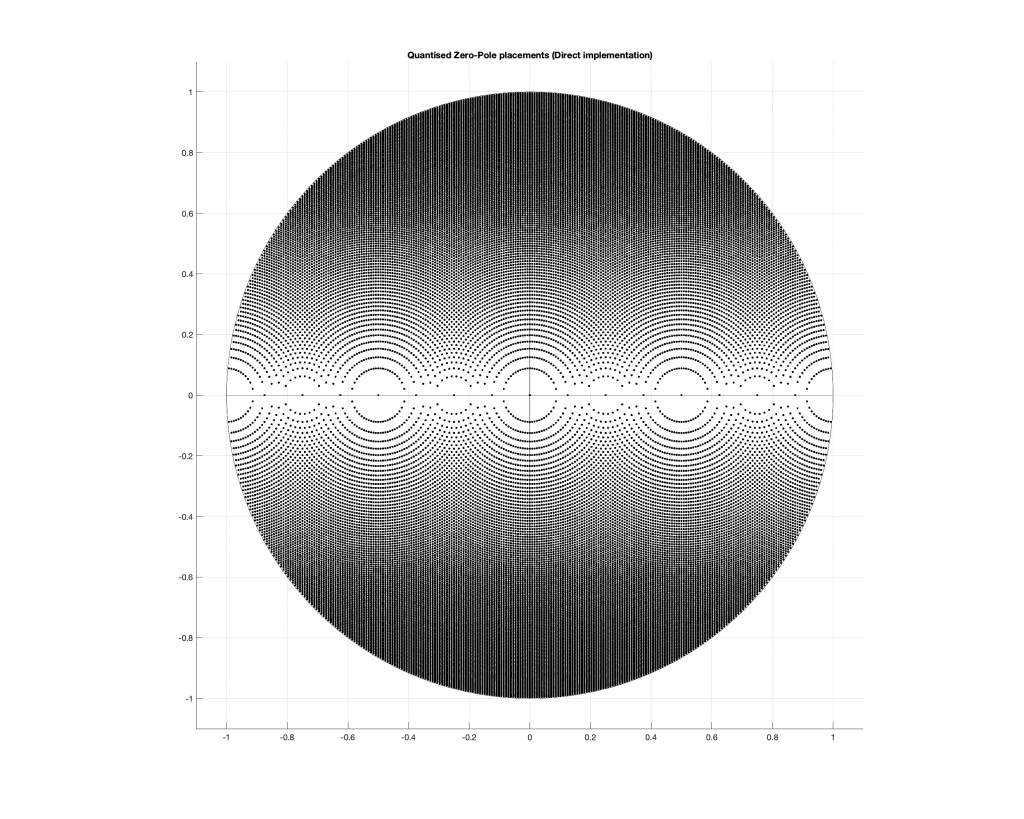Figure 6: The dots indicate the possible locations of the poles as a result of quantisation of the gain coefficients in a 4-bit Direct Form implementation.

Any higher than this and the pattern will just get so dense that it’ll turn black, so I’ll stop.

## So what?

At this point, you may be asking “so what?” The answer to this very important question lies on the far right side of all of those graphs. Notice that there aren’t any locations near the point on the graph where X=1 and Y=0. You may remember from Figure 7 in this posting that I did last week, that that’s the point on the Z-plane that corresponds to very low frequencies.

This means that, if you want to create a digital filter, and you need a pole near 0 Hz, you’re going to run into some trouble with a Direct Form implementation of the biquad. Yes, the higher the bit depth of the gain coefficients, the closer you can get, but this might not be the best way to do things.

There is another option for implementing your feed-back portion of the biquad. You can use a design called a Coupled Form instead, which is shown in Figure 7 (compare it to Figure 3).

Notice that you still have two 1-sample delays, however there are now 4 gains instead of 2. How can this be better? Well, in a system with infinite resolution on the gain coefficients, it’s not. Given the appropriate choice of gain values, this implementation will do exactly the same thing as the Direct Form implementation if your resolution is infinite.

However, if you have a limited resolution, then the available locations of the poles on the Z-plane are very different. Let’s use the 4-bit, 6-bit, and 8-bit quantisations of the gain values again: these are shown in Figures 8 to 10.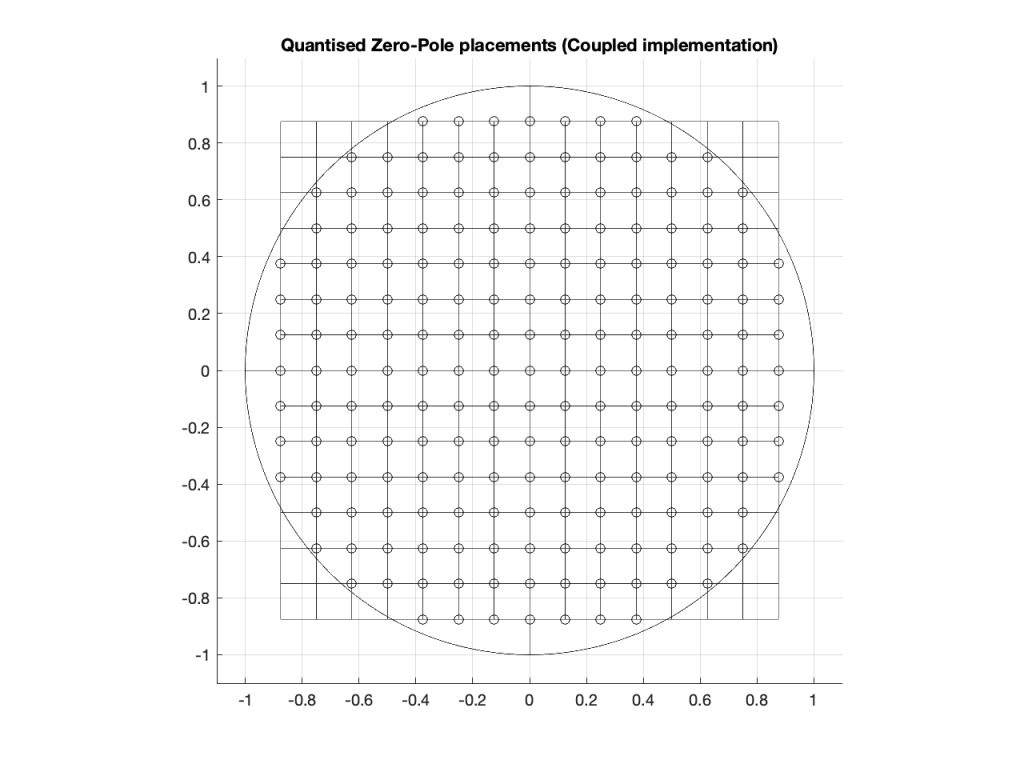Figure 8: The circles indicate the possible locations of the poles as a result of quantisation of the gain coefficients in a 4-bit Coupled Form implementation.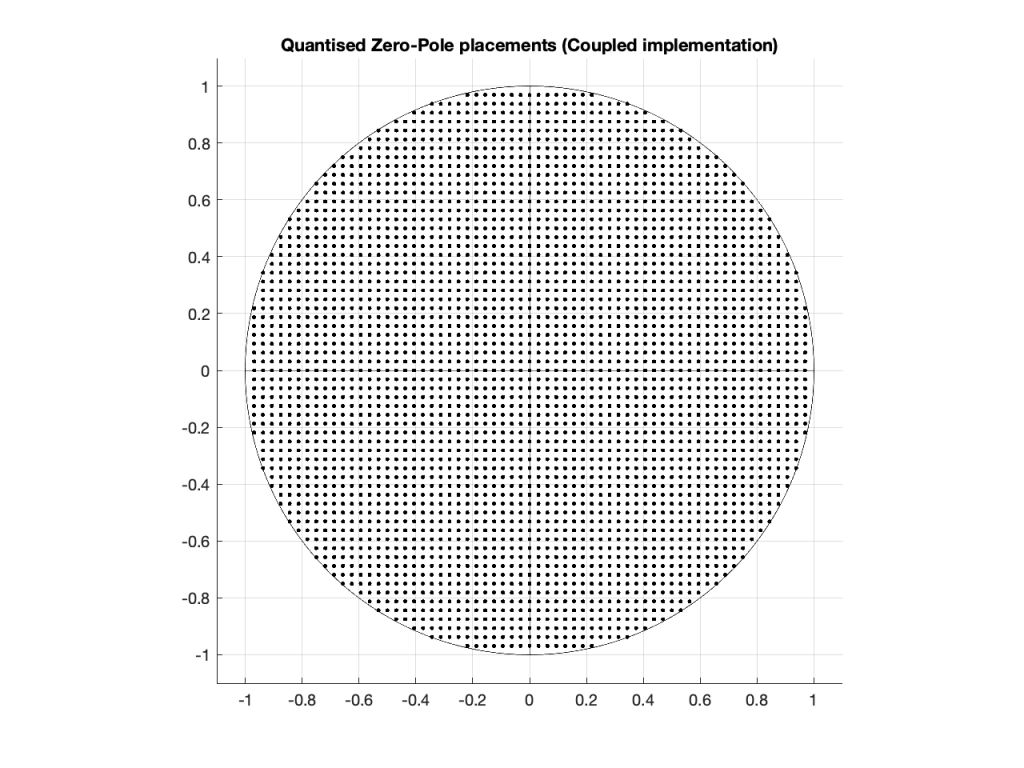Figure 9: The dots indicate the possible locations of the poles as a result of quantisation of the gain coefficients in a 6-bit Coupled Form implementation.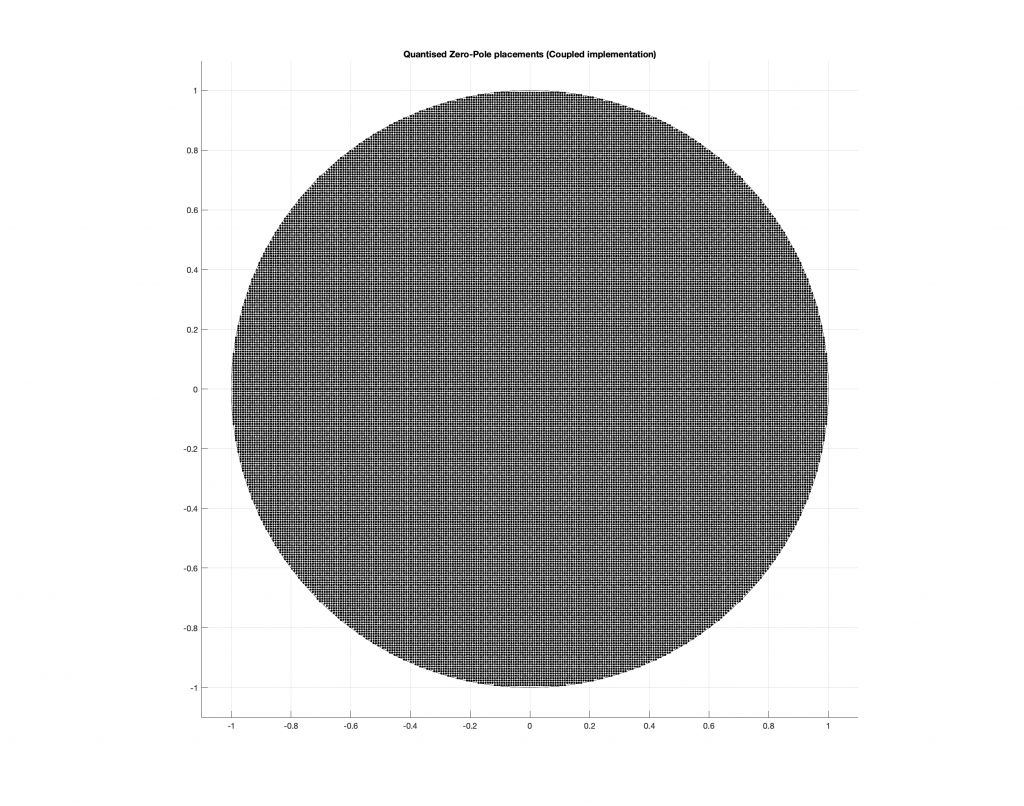Figure 10: The dots indicate the possible locations of the poles as a result of quantisation of the gain coefficients in a 8-bit Direct Form implementation.

As you can see in Figures 8 through 10, the Coupled Form implementation, given the same resolution on the gain coefficients, will give you much better placement opportunities for the poles in the low frequency region than the Direct Form implementation.

Of course, in all of these examples, I’m only showing up to an 8-bit word, and a typical DSP runs uses a lot more than 8 bits for the gain coefficients. So, it’s possible that, in the real world, the actual resolution is high enough that this is of no concern whatsoever.

However, if you’re building a very-low-frequency filter an if the magnitude response isn’t exactly what you’re looking for, this might help you get a little closer to your goal.

It’s important to point out here that the quantisation of the locations of the zeros is not the same as this. Someday, I’ll come back to this and plot the expected vs. actual magnitude responses of some biquads where I’ve quantised the zeros and poles, just to see how badly things go wrong when they do…

This posting is a simple summary of the discussion of a section called “Poles of Quantized Second-Order Sections” in “Discrete-Time Signal Processing” by Alan V. Oppenheim and Ronald W. Schafer.

The Coupled Form implementation was introduced by C.M. Rader and B. Gold in their paper called “Digital Filter Design Techniques in the Frequency Domain” from the Proceesings of the IEEE, Vol. 55, pp. 149 – 171 , from February, 1967.

# Intuitive Z-plane: Part 5 – Conclusion

If you’ve read through the first four parts of this series, then you’re already at a point where you can intuitively understand what’s going on. We just have a couple of details to take care of before finishing off.

Firstly, the plots showing the zeros and poles in the figures you’ve been looking at plots of the “Z-plane” or “Complex-plane“. As I said at the start, we’re only trying to get to an intuitive understanding of these plots – so I’m not going to get into complex numbers, or even much math (apart from what you’ll see below… which isn’t very complicated, and avoids complex numbers).

When I’m developing a new DSP algorithm, I use an application called Max from cycling74.com. Figure 1 shows a screenshot from Max, where I’m using an object to calculate the biquad coefficients to make a low pass filter, as you can see. I’ve then connected the output of that object (it looks like a magnitude response) to a Z-plan representation that shows me the same thing in a different way.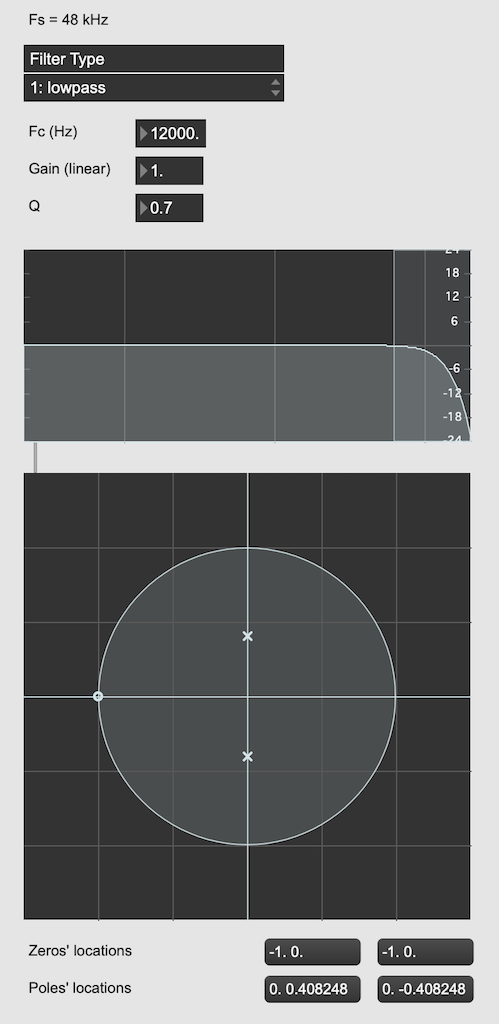Figure 1: The top plot shows the magnitude response of the filter. The bottom plot shows the Z-plane representation of the same filter.

You may notice that this plot has two poles, one at (0, 0.408) and the other at (0, -0.408). In fact there are two zeros there as well, but they’re situated in the same place, on “on top” of the other, at (-1, 0). This is always true for a biquad – there are always two zeros and two poles. Sometimes, they’re located in the same place, sometimes not, sometimes they’re placed symmetrically, sometimes not, depending on the filter, as we’ll see below.

Let’s look at that Z-plane representation in 3-dimensions: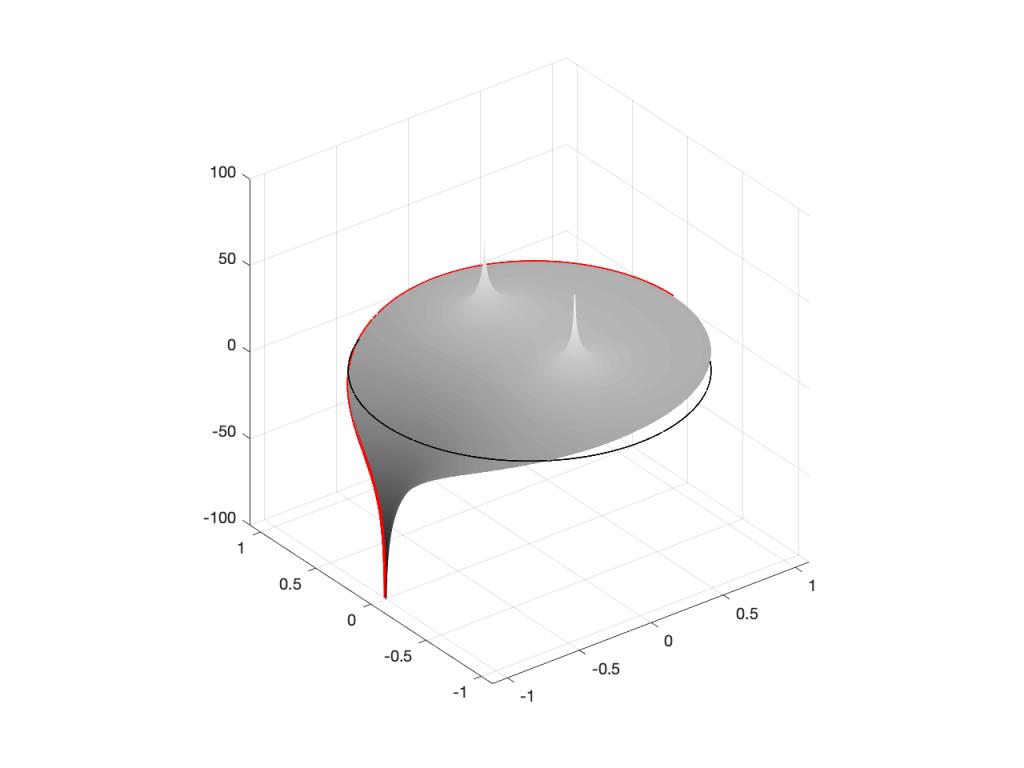Figure 2: A 3D view of the Z-plane representation of the filter shown in Figure 1.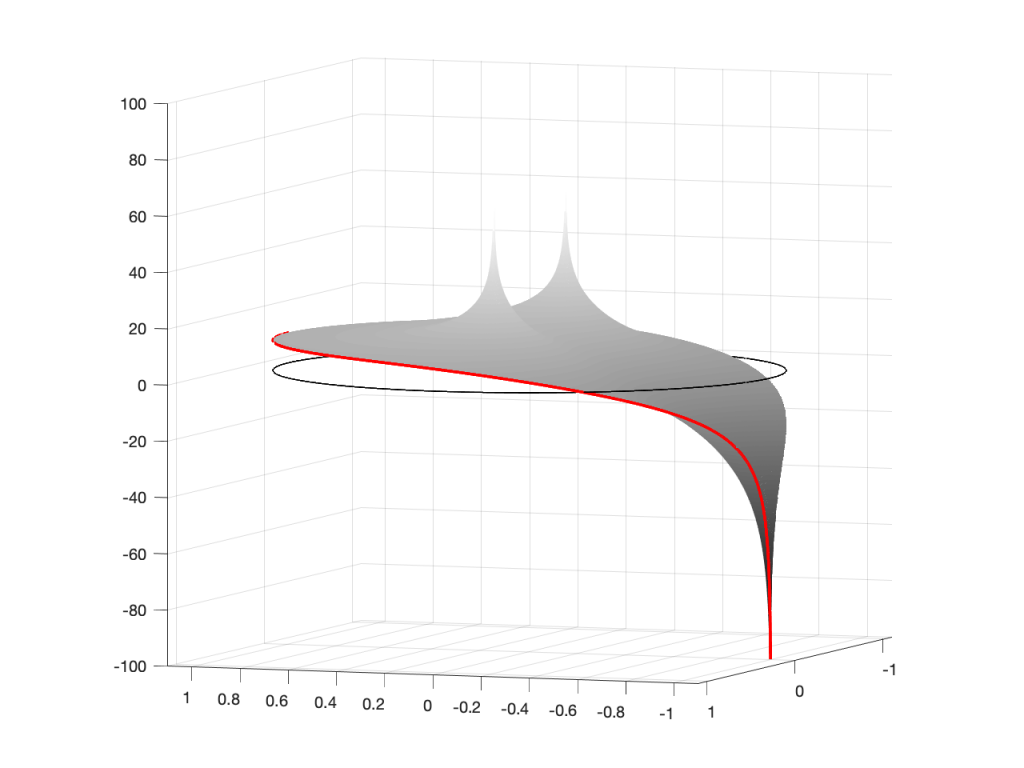Figure 3: The same plot as shown in Figure 2, rotated to show the back of the plot.

So, as you would now expect, the poles pull up the edge of the circle, and the zeros (both in the same place) pull down, giving the red line the height that it has.

Now, think back to this Figure from earlier in the series:

If you therefore look at Figure 3, which is like looking at Figure 4 from the top, you’ll notice that the height of the red line (the edge of the circle is high on the left (in the low frequencies) and drops as you go to the right (the high frequencies). This is the magnitude response that’s shown on the top of Figure 1. The only difference is that it’s on a linear scale instead of a logarithmic scale, so the shape looks a little weird.

Let’s do another one: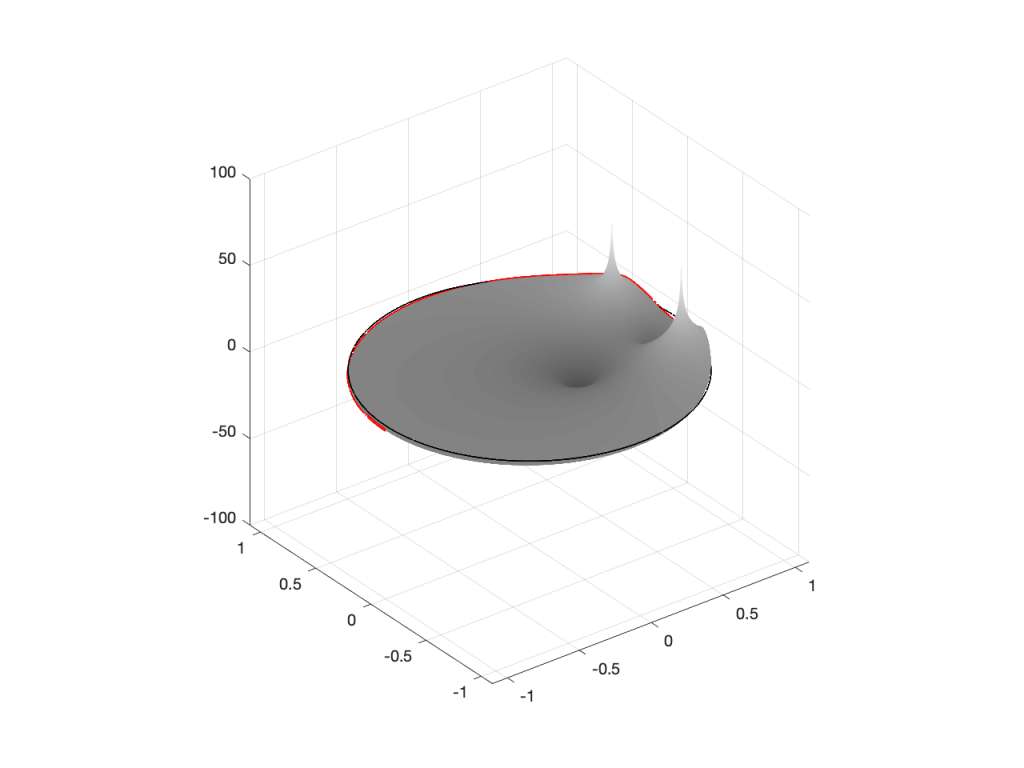Figure 6: A 3D view of the Z-plane representation of the filter shown in Figure 5.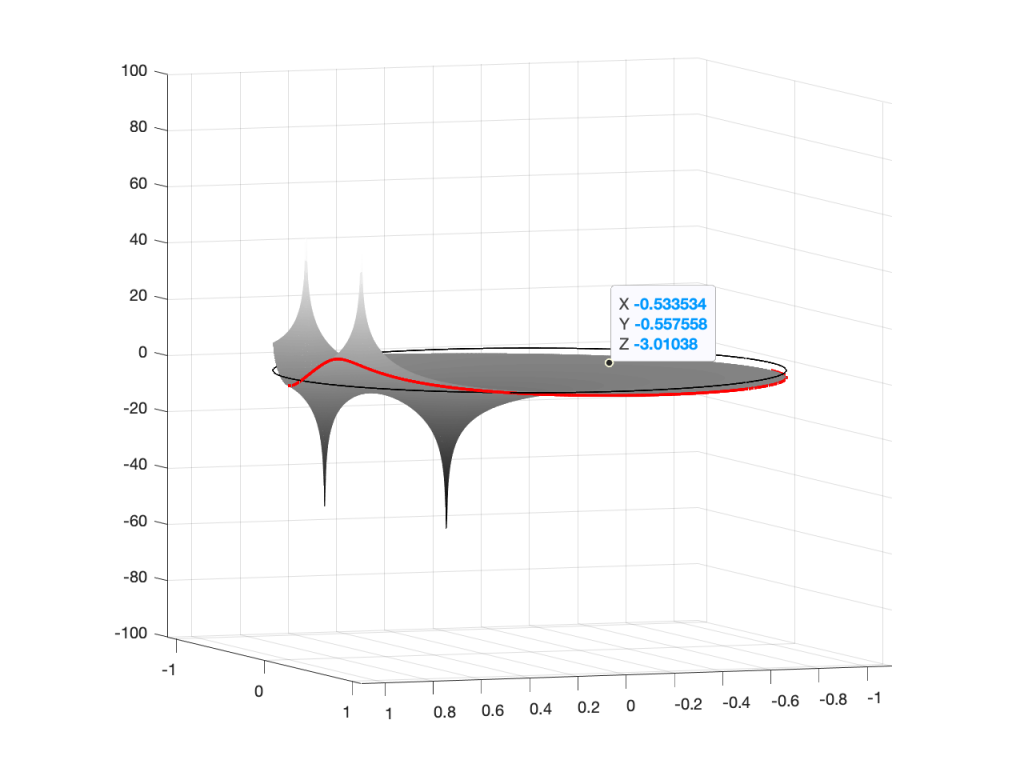Figure 7: The same plot as shown in Figure 6, rotated to show the back of the plot.

Hopefully, now you are able to look at a Z-plane representation of a filter and think about the effect of the poles and zeros on the edge of the circle, and therefore get a rough idea of the magnitude response of the filter…

If not, I apologize for wasting your time. On the other hand, if you’re in a life-threatening situation, this knowledge probably wouldn’t help you anyway… Very few people have gotten a critical injury in a biquad accident.

## How I did it

If you want to make these plots for yourself, the math is pretty simple.